# RD Sharma Solutions for Class 9 Maths Chapter 4 Algebraic Identities

## RD Sharma Solutions for Class 9 Maths Chapter 4 – Free PDF Download

RD Sharma Solutions for Class 9 Maths Chapter 4 – Algebraic Identities is the most popular study material among students. The answers to all the textbook questions are curated by subject experts at BYJU’S in a comprehensive manner. For more conceptual knowledge students can follow these solutions designed by experts in a simple language. The algebraic equations which are true for all values of variables in them are called algebraic identities. RD Sharma Class 9 Solutions are helpful to obtain an idea of important questions that might be asked in board exams. Thorough practice of these solutions boost time management and logical thinking abilities among students.

Students who aim to secure good marks in their board examinations can refer to RD Sharma Solutions. These solutions are formulated by the BYJU’S expert team to help students understand the covered concepts in an easy manner. Students who face difficulty in solving exercise wise problems of RD Sharma textbook can access solutions in a PDF format both online and offline mode.

## Download PDF of RD Sharma Solutions for Class 9 Maths Chapter 4 Algebraic Identities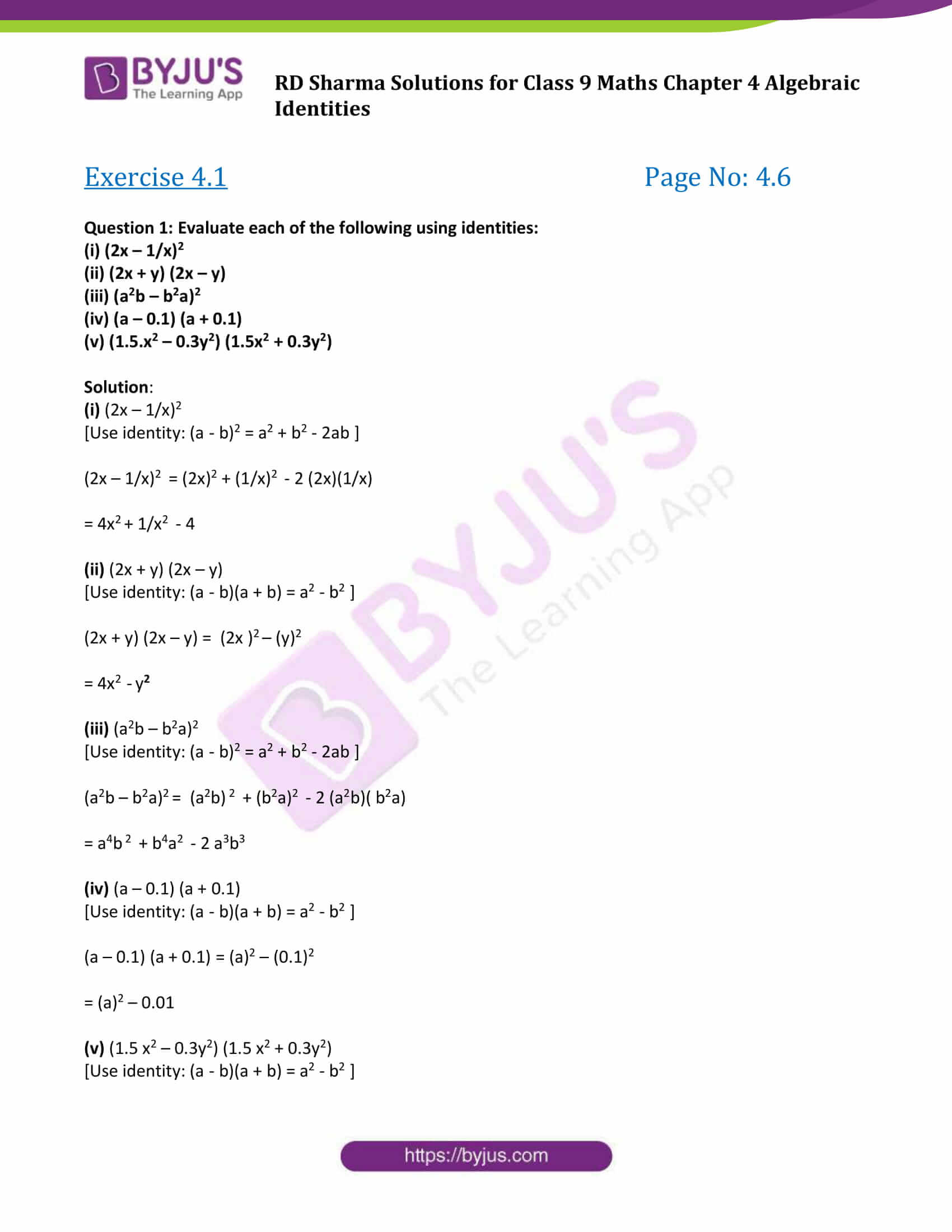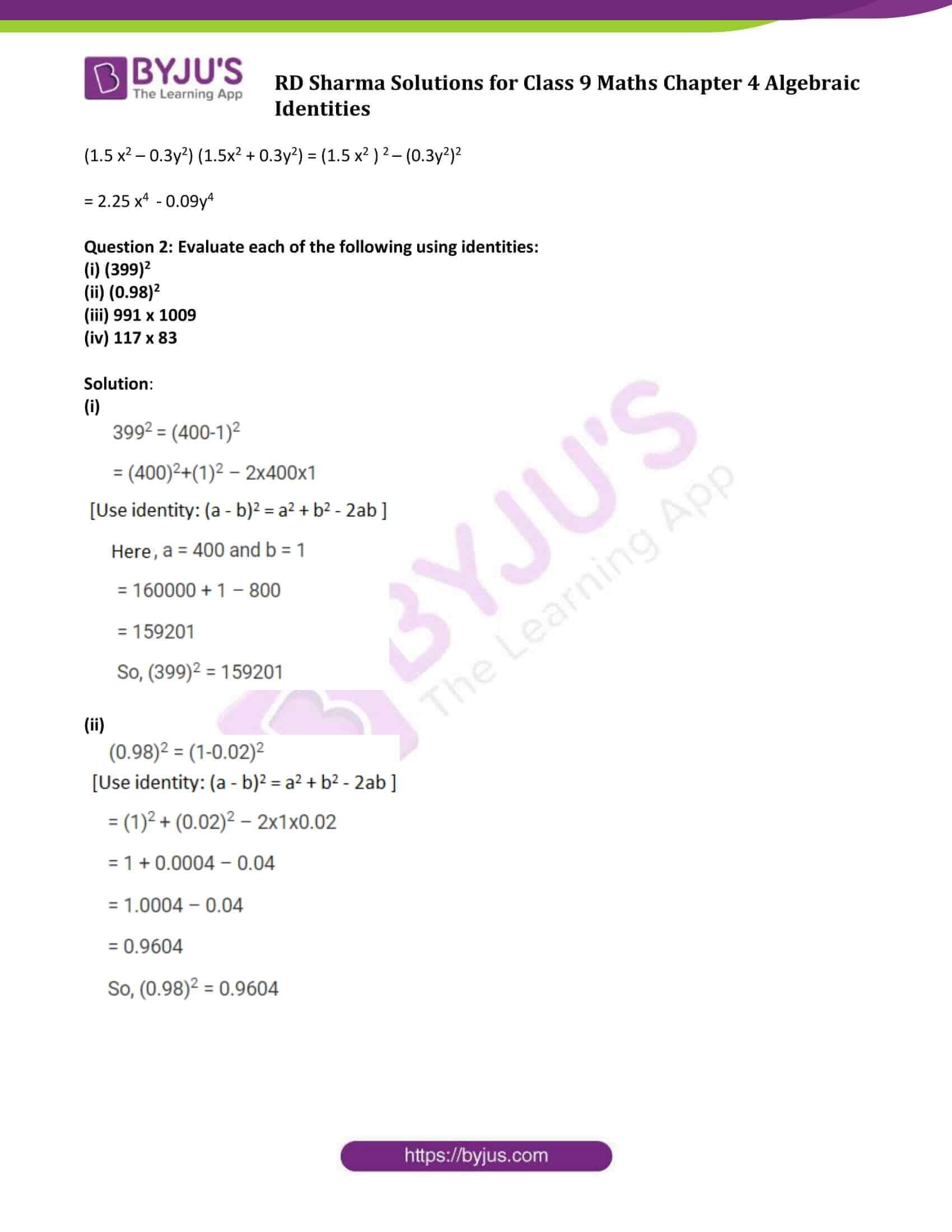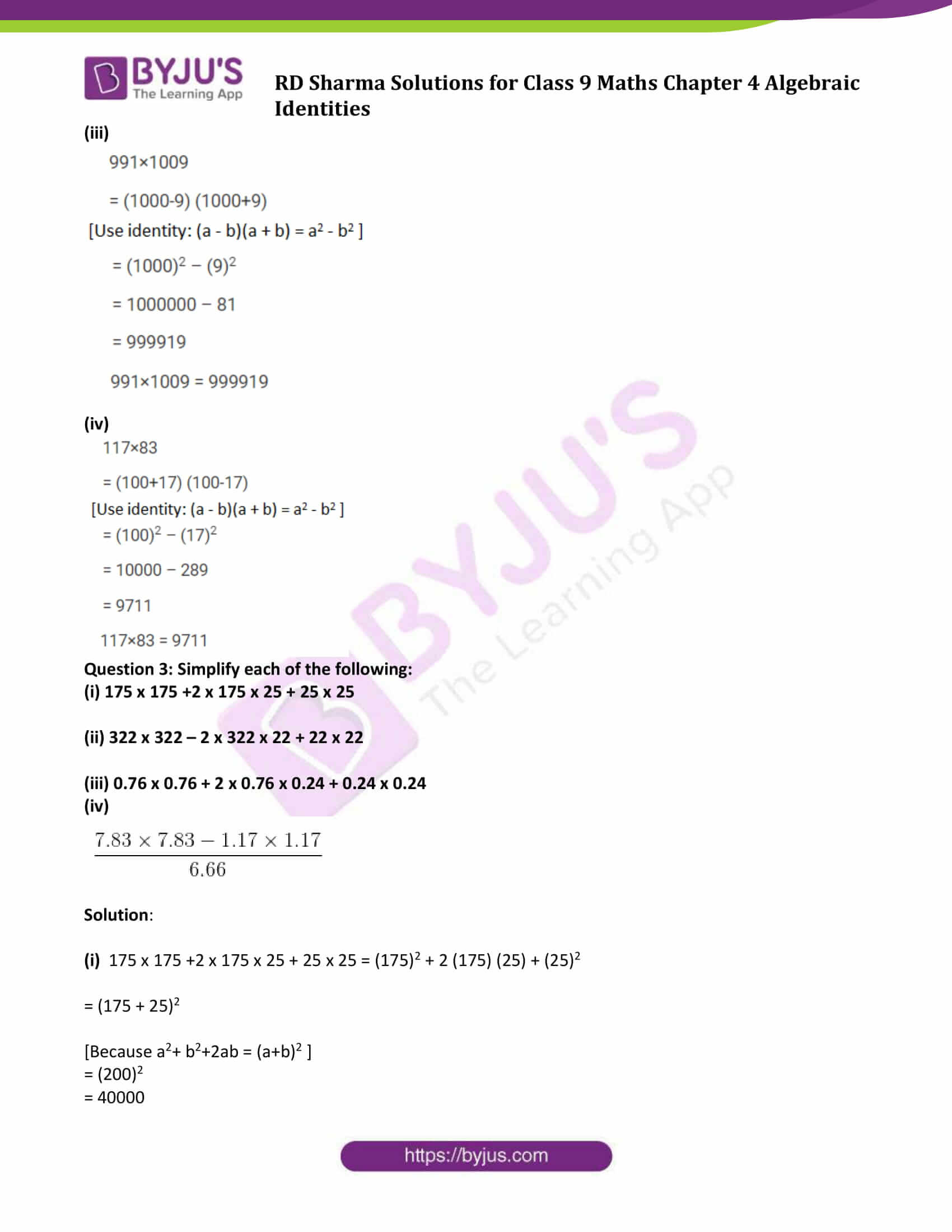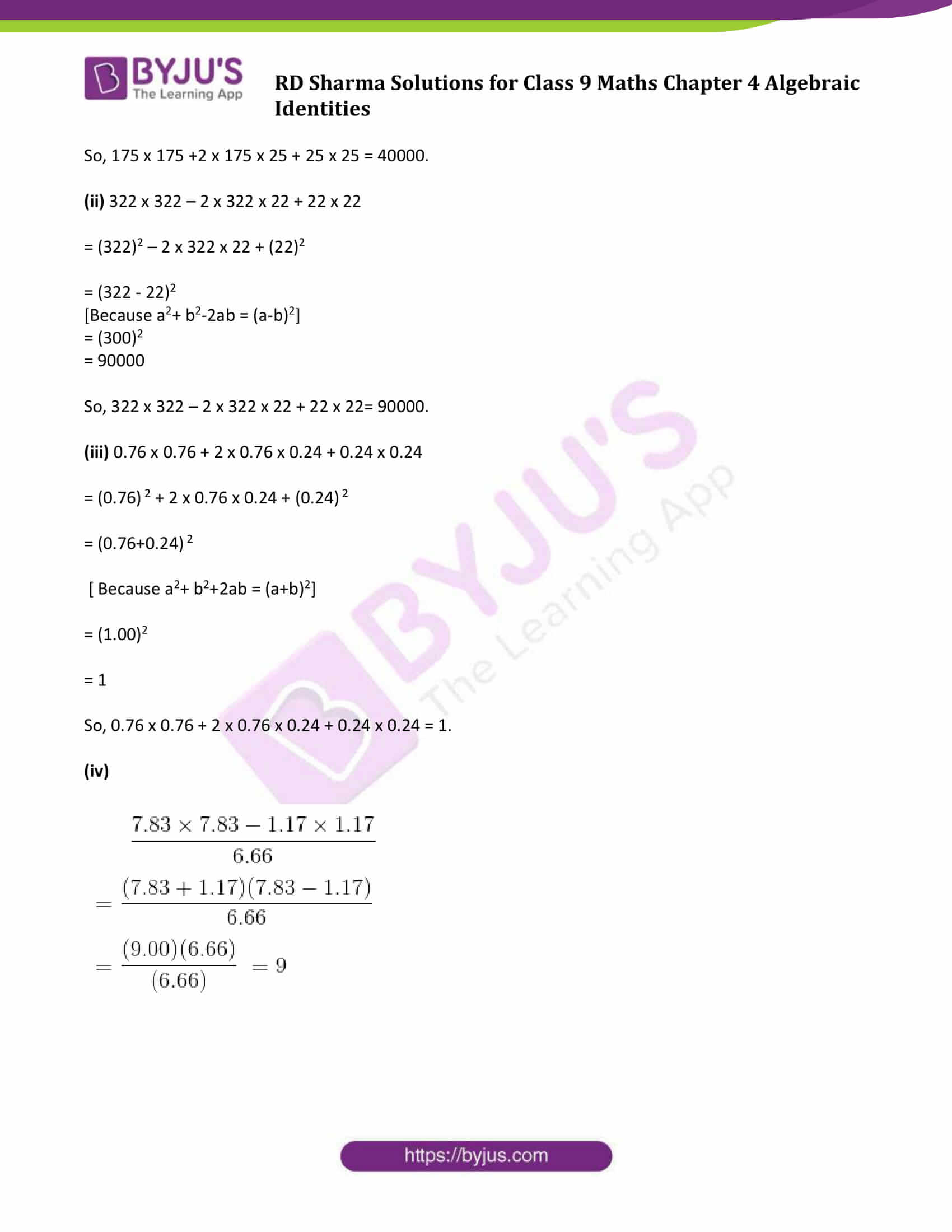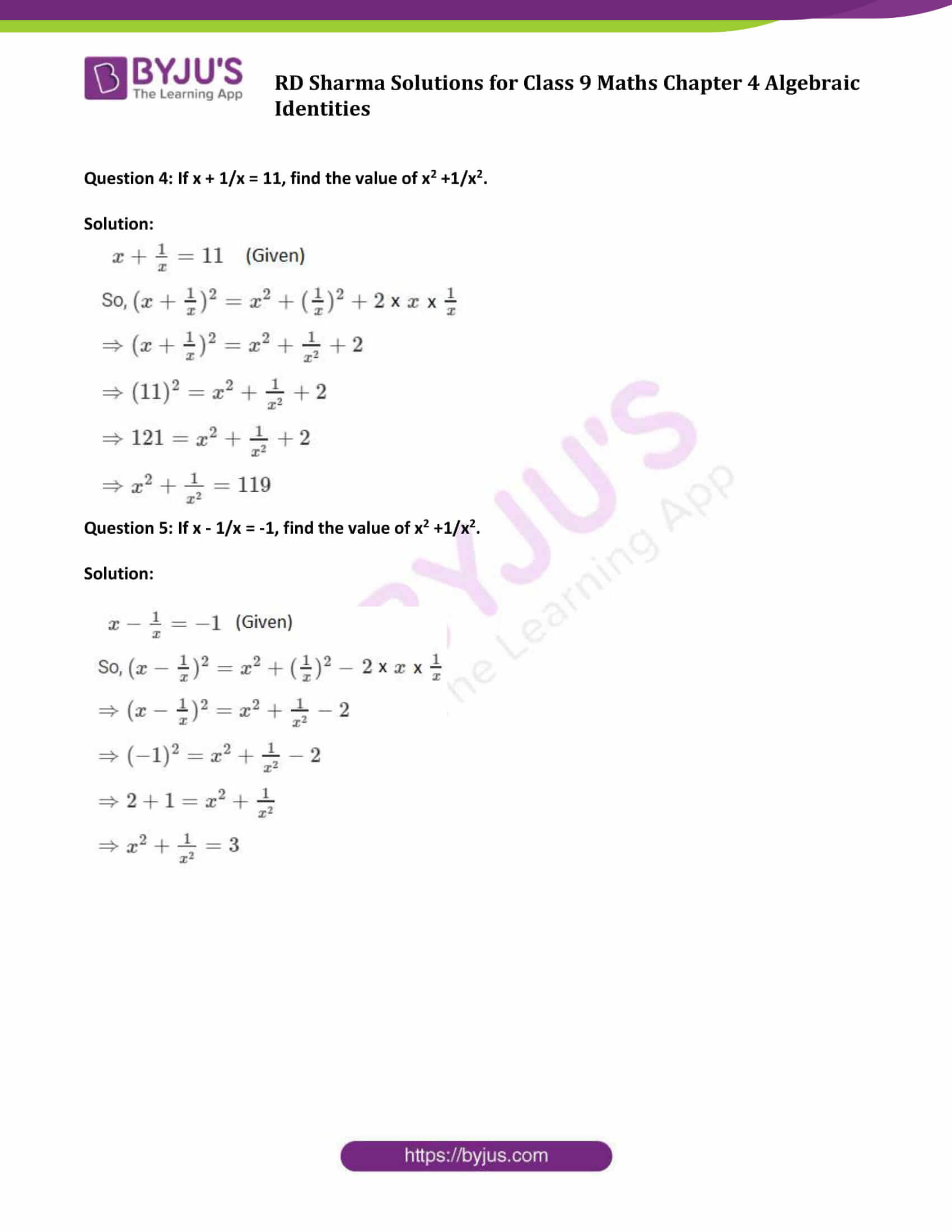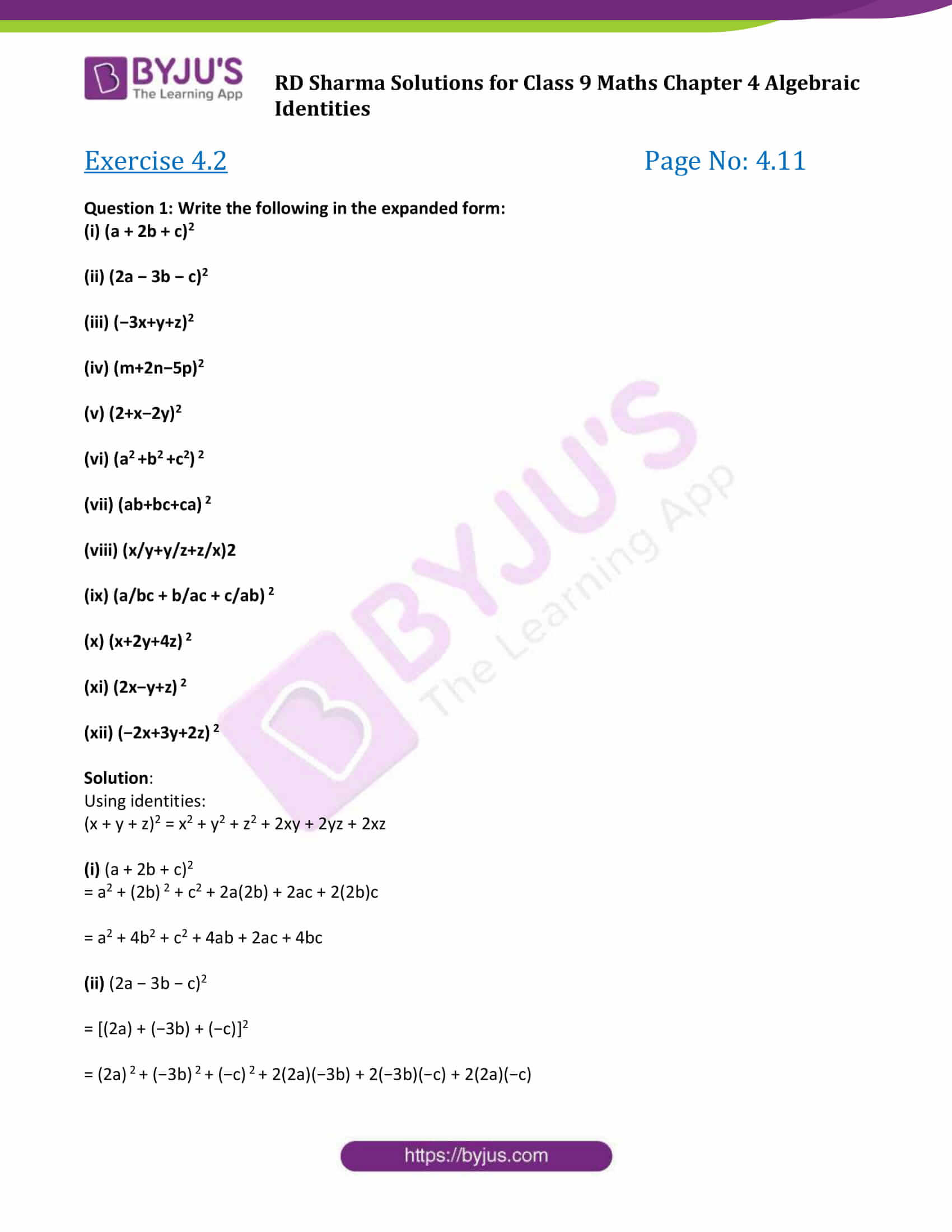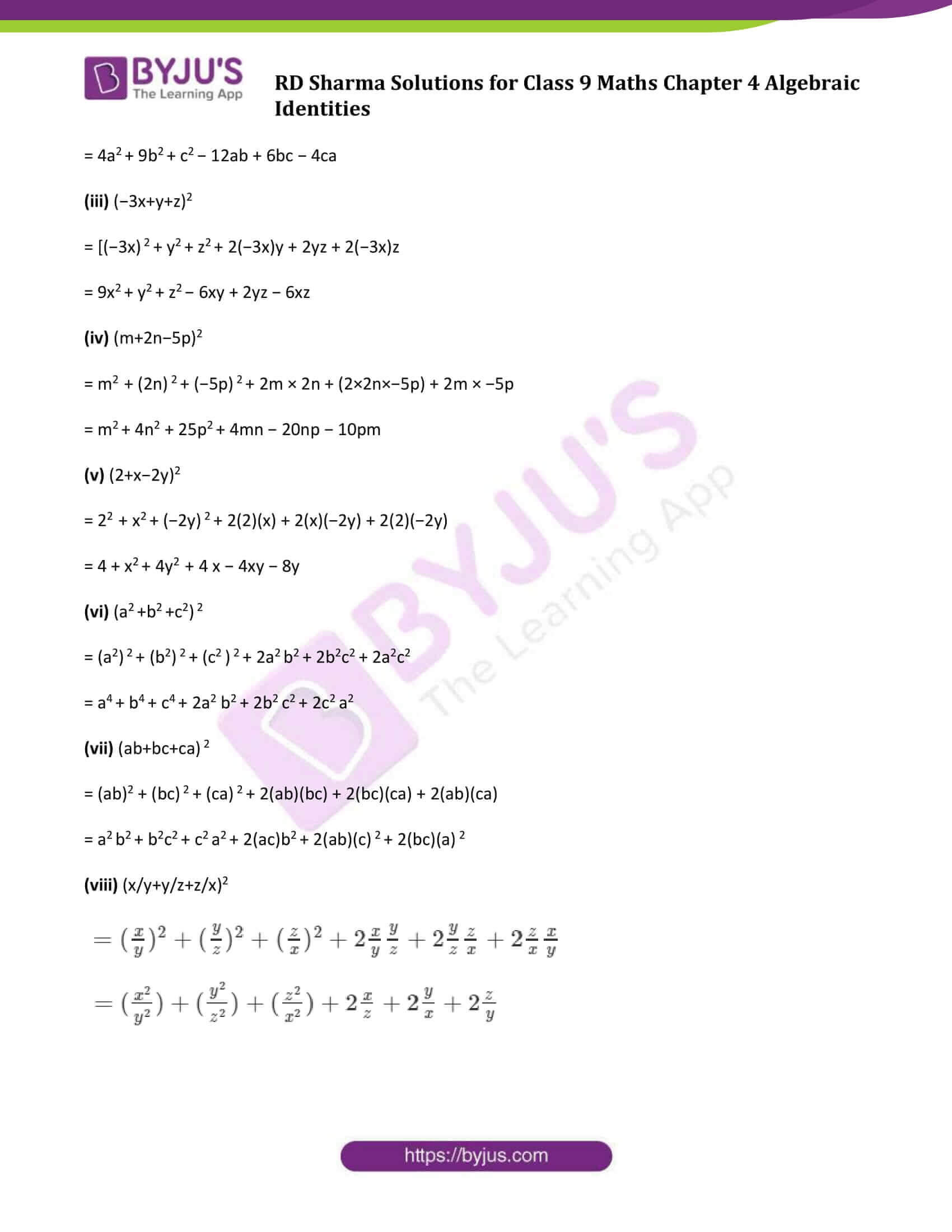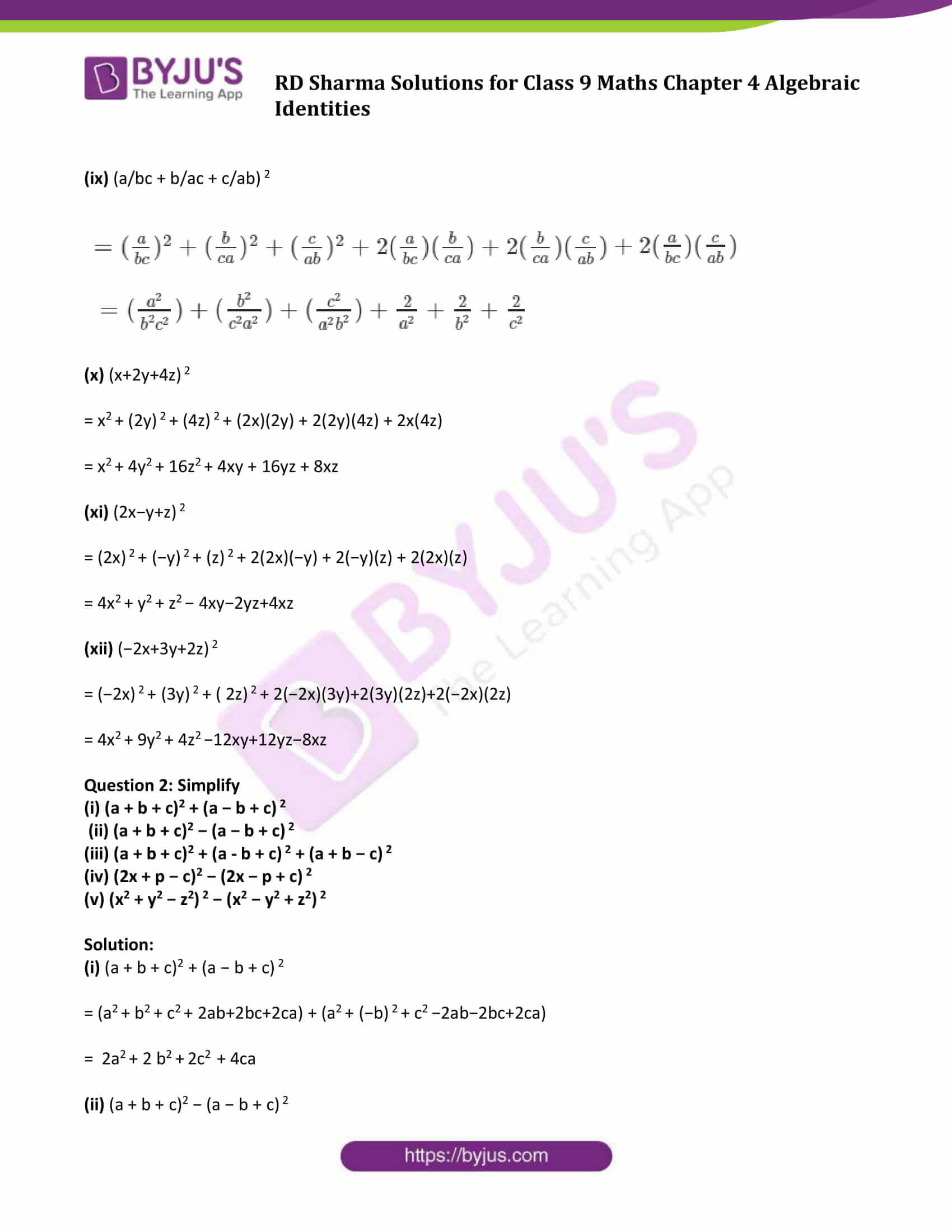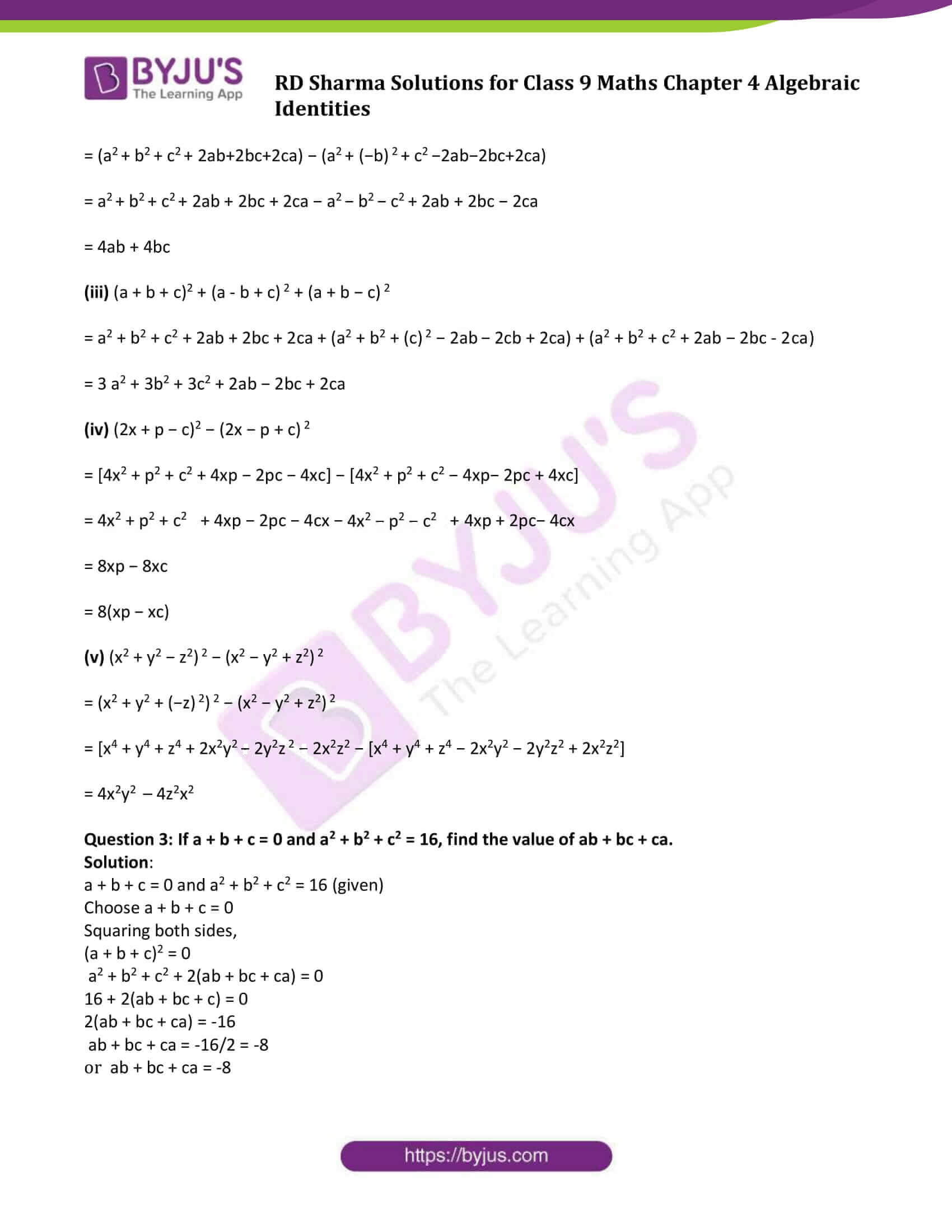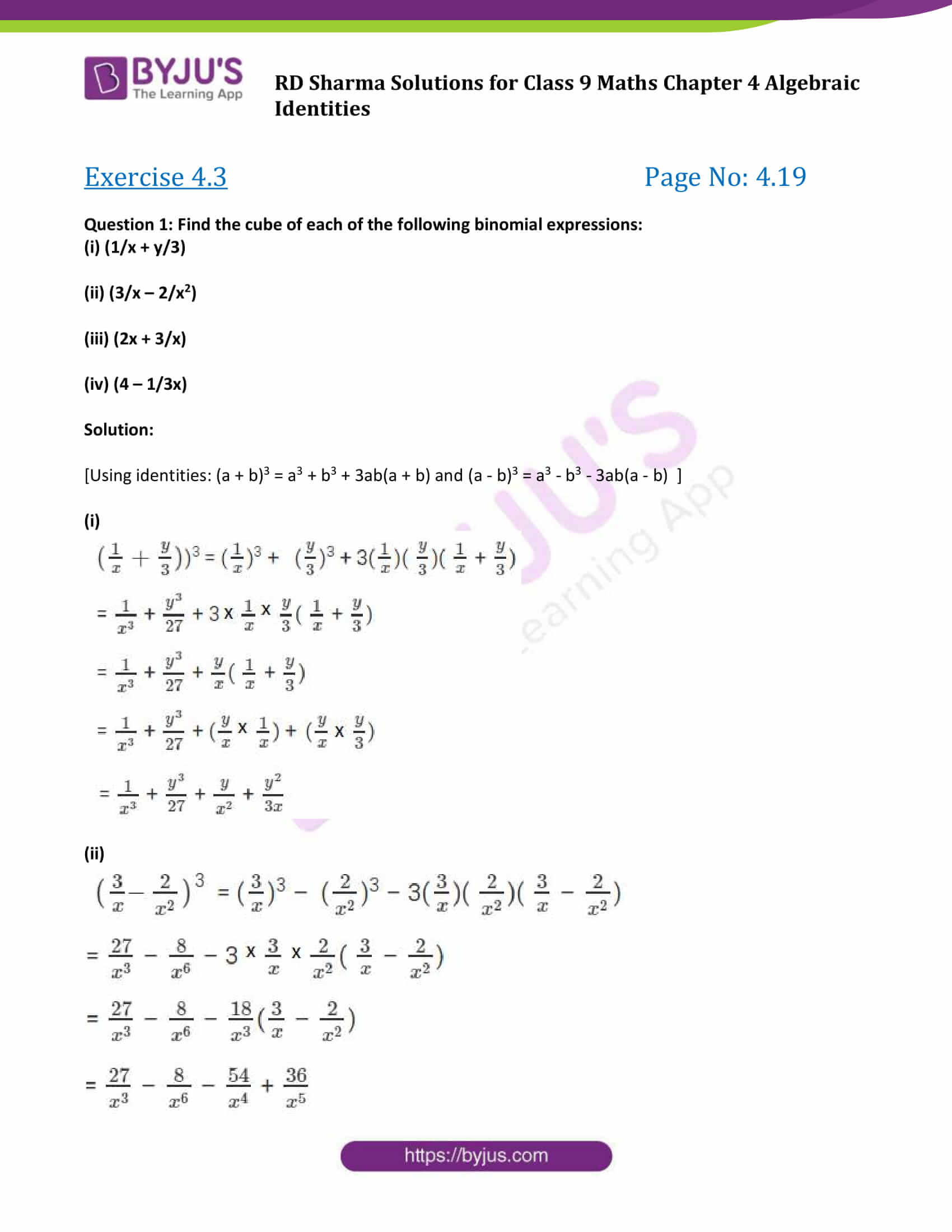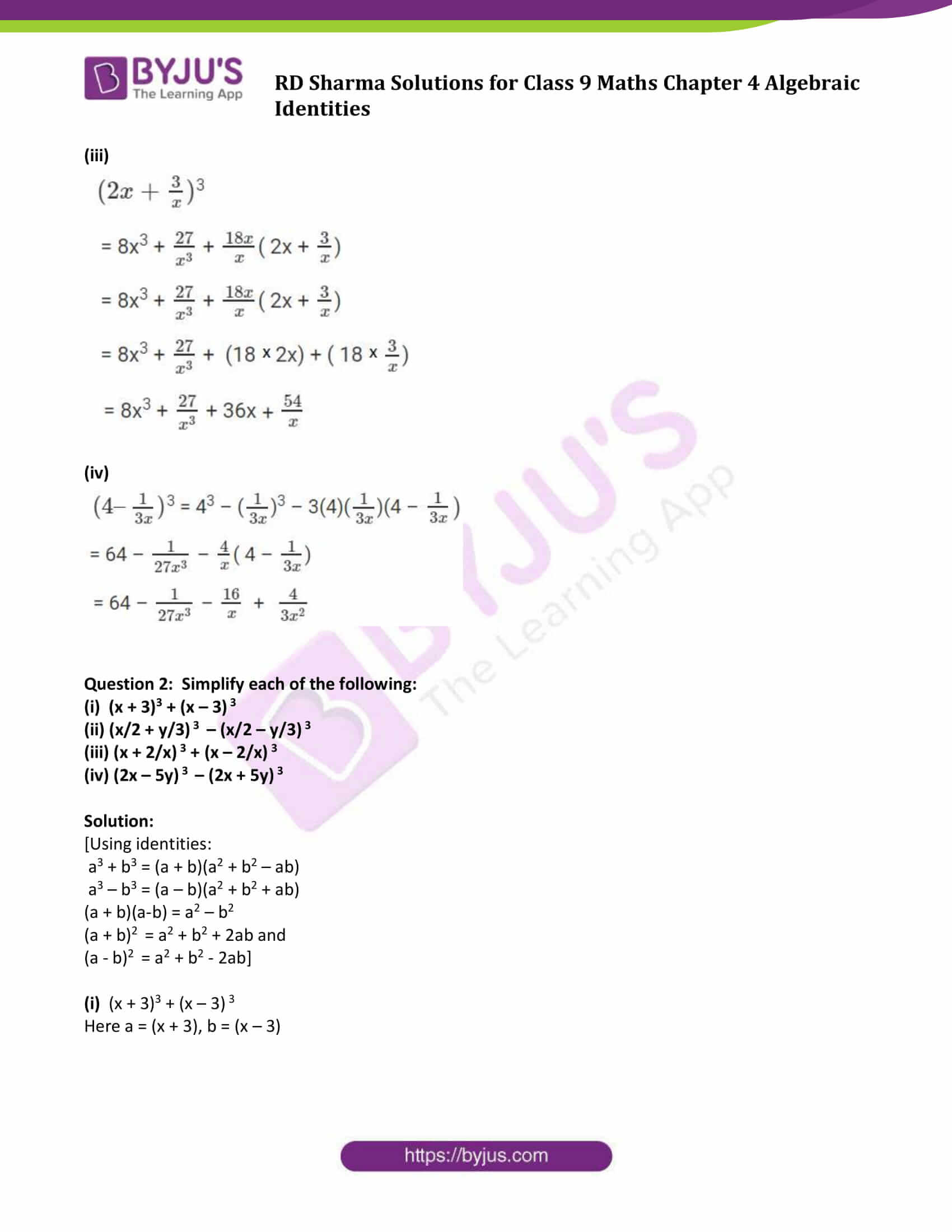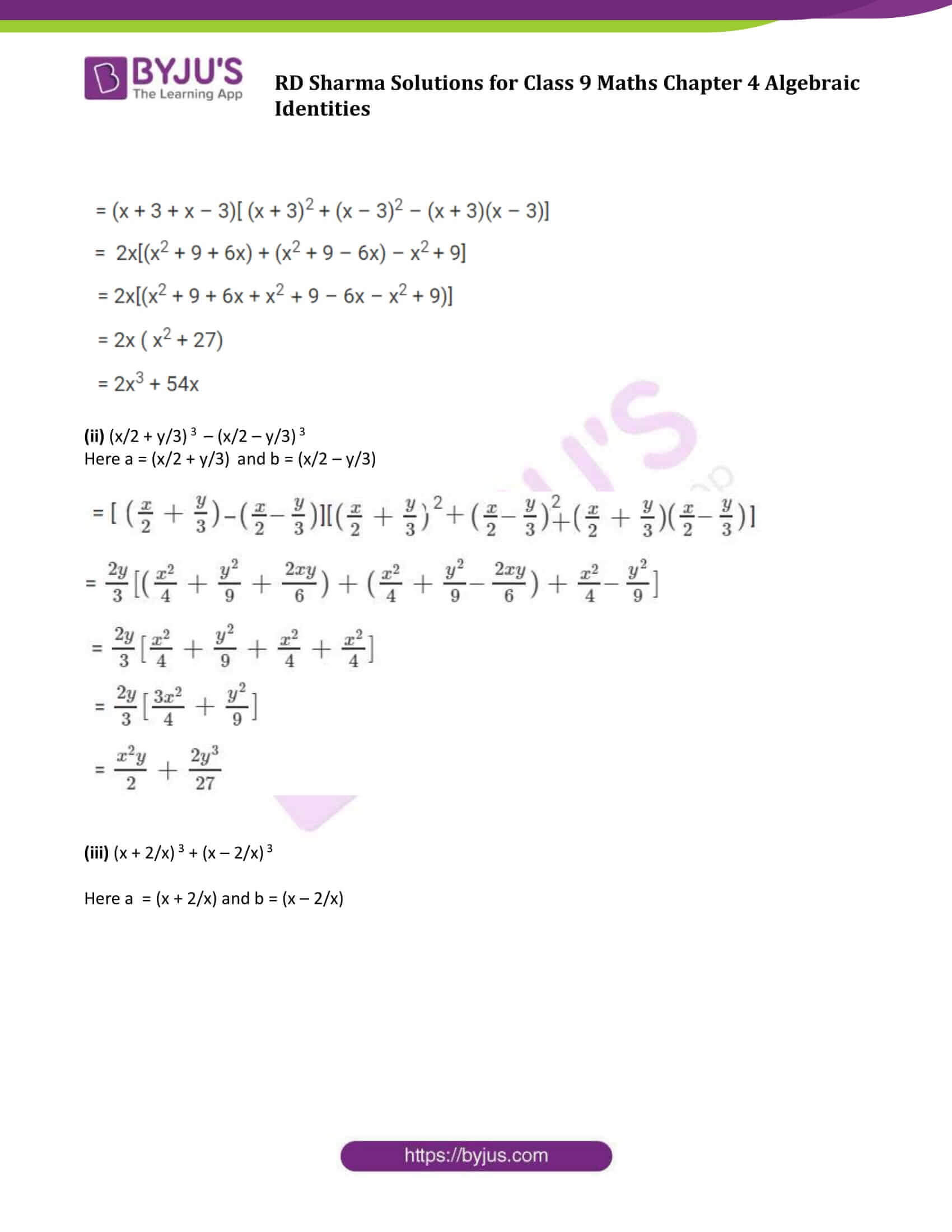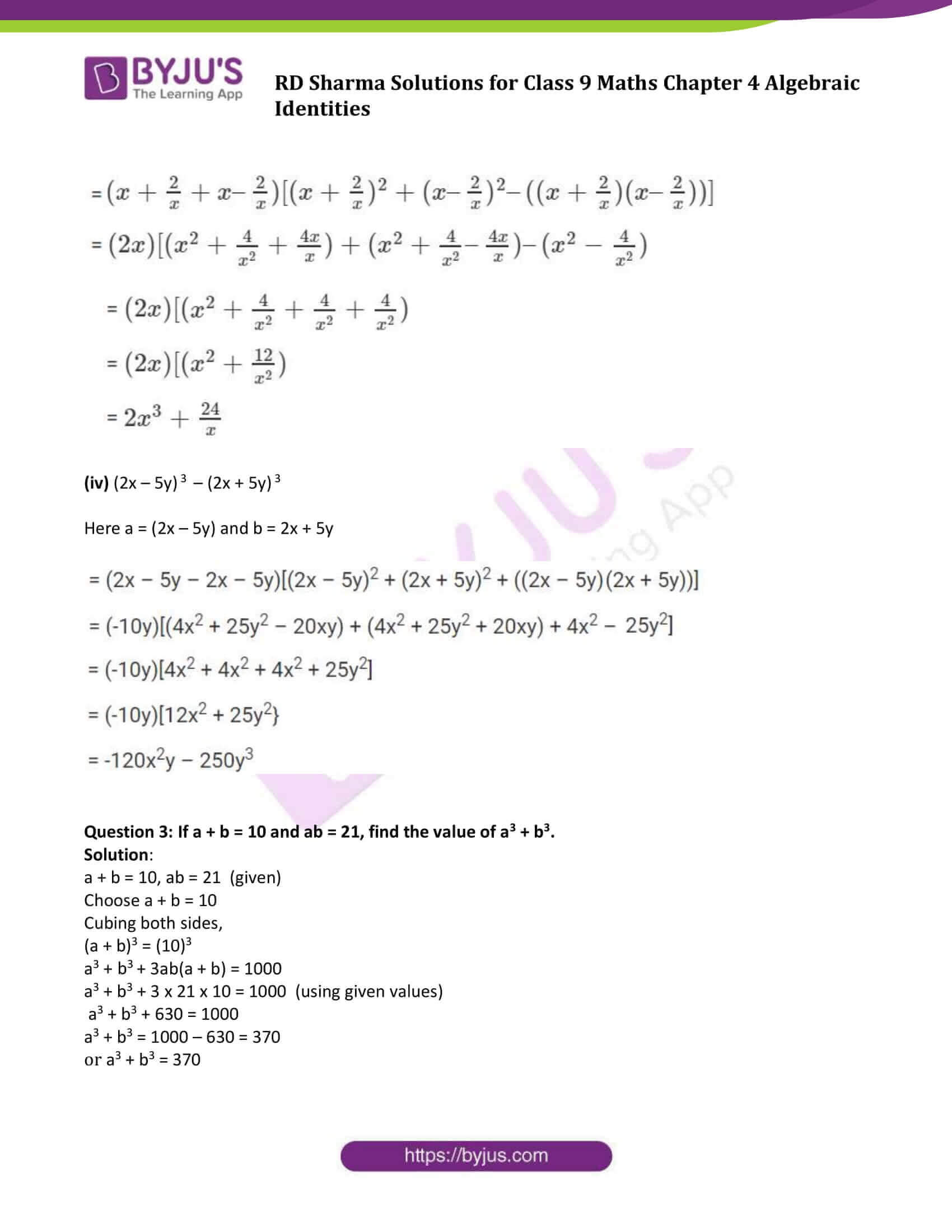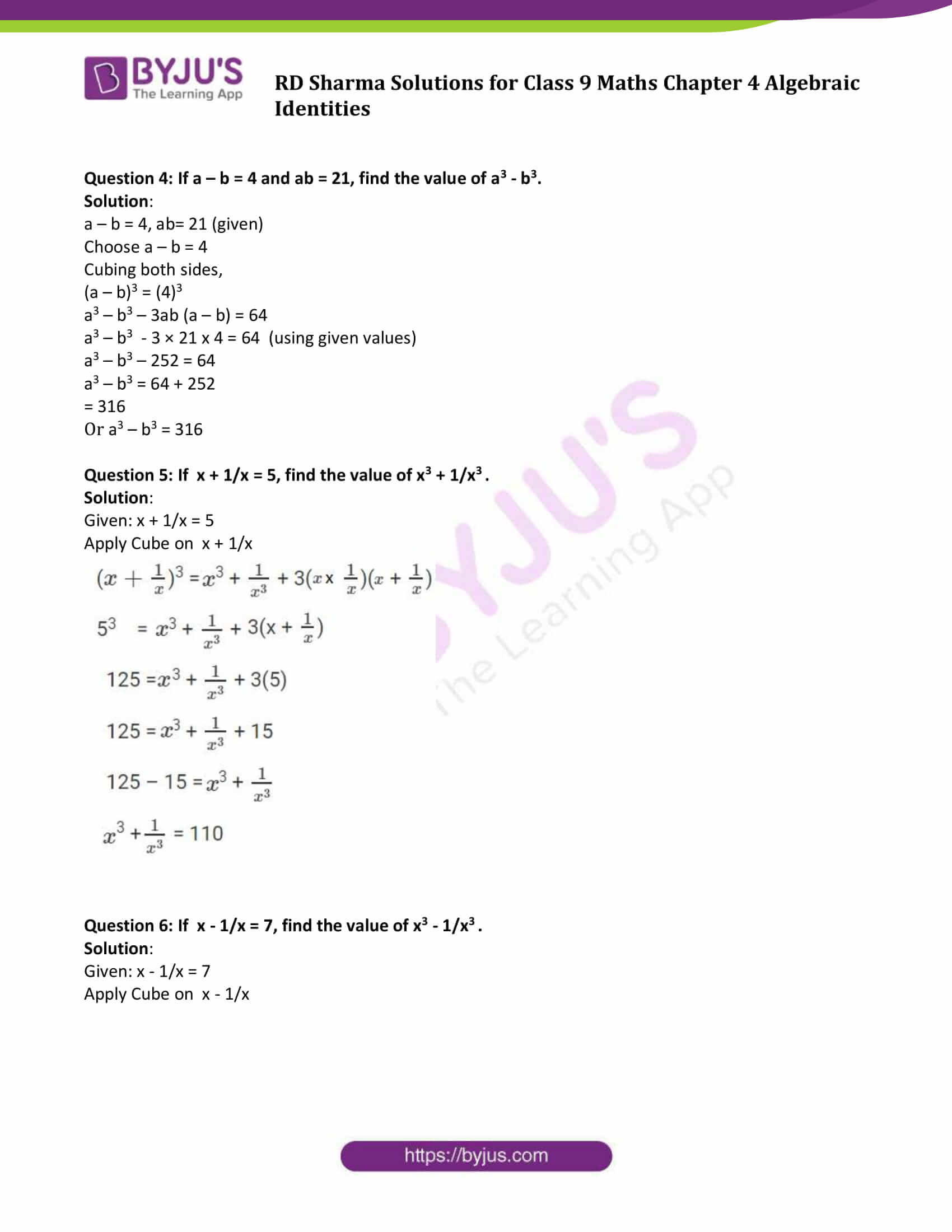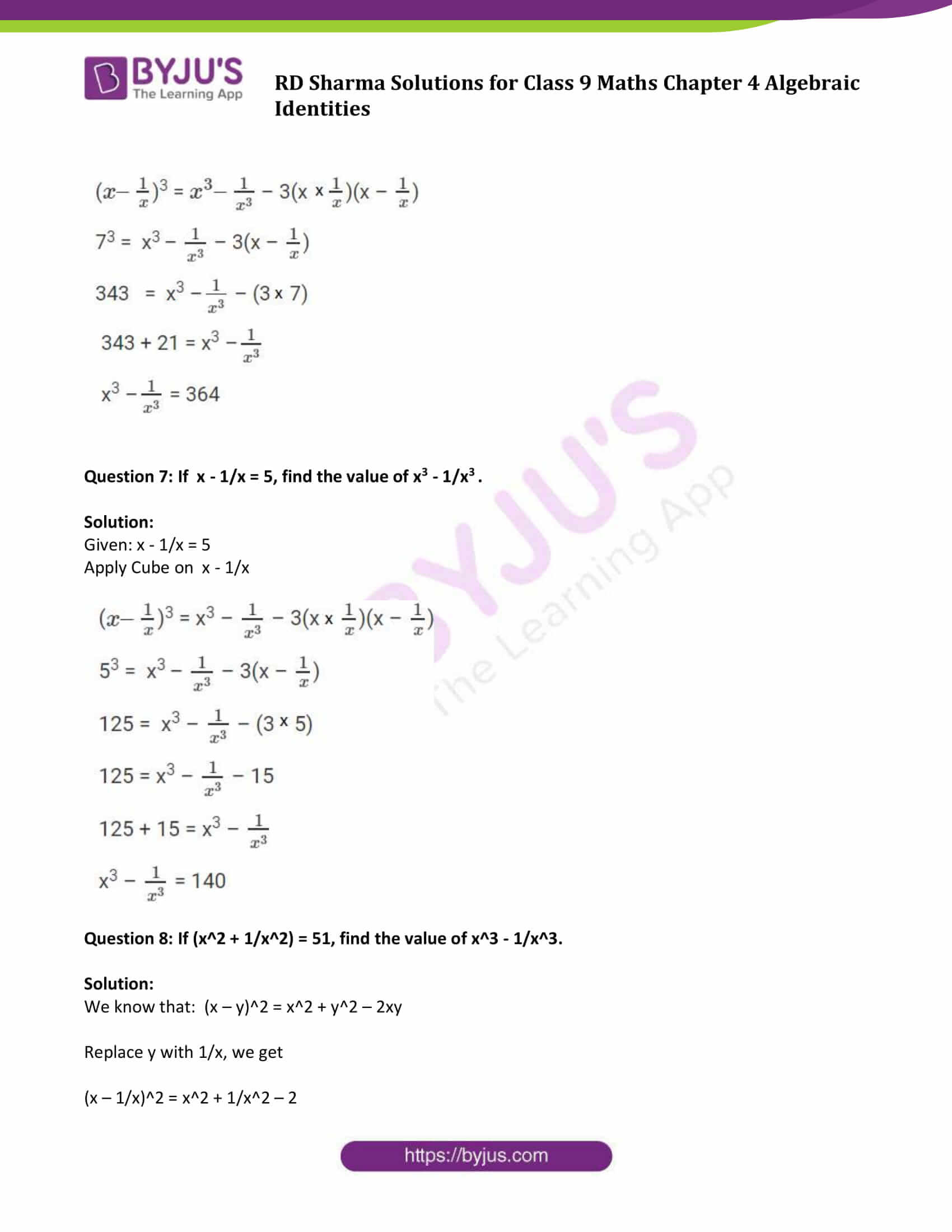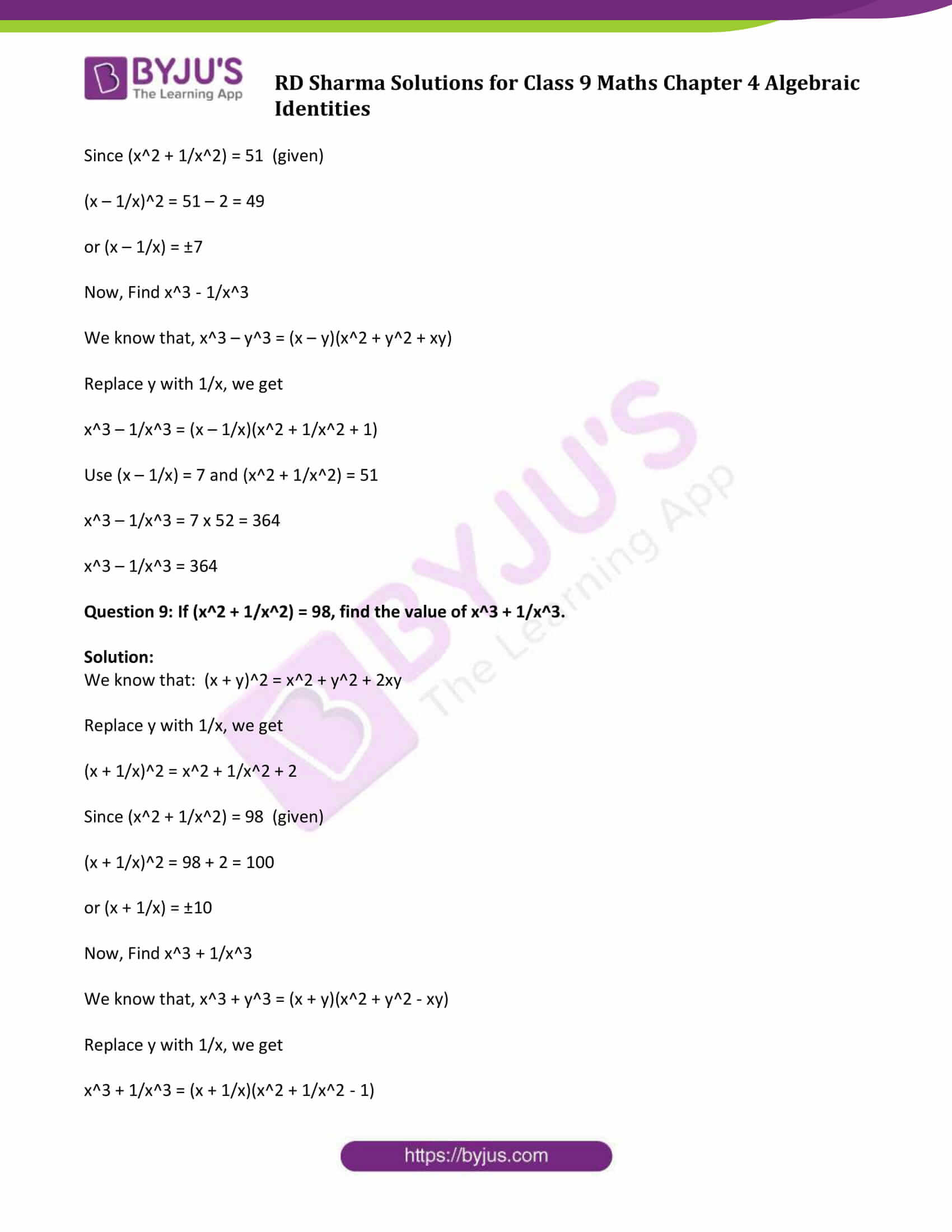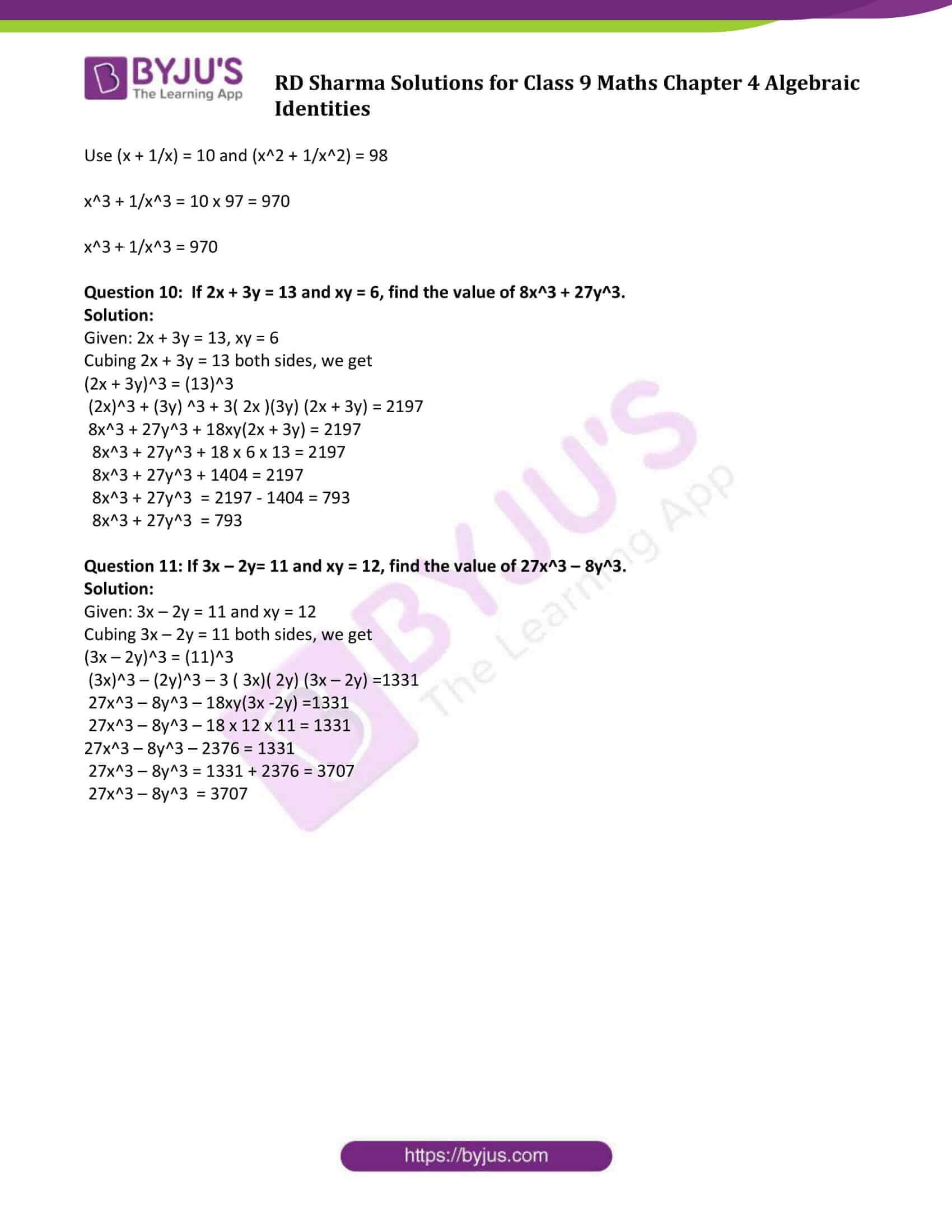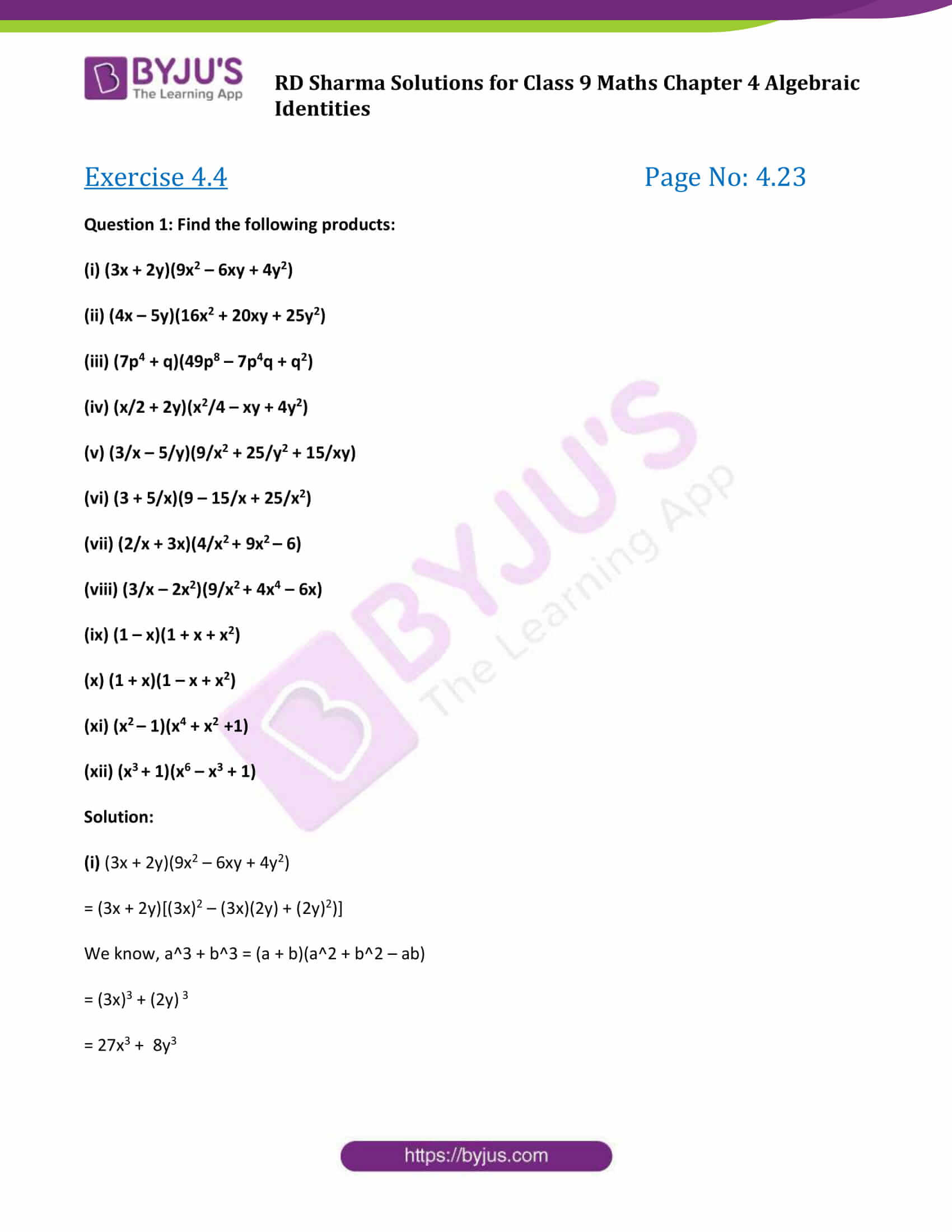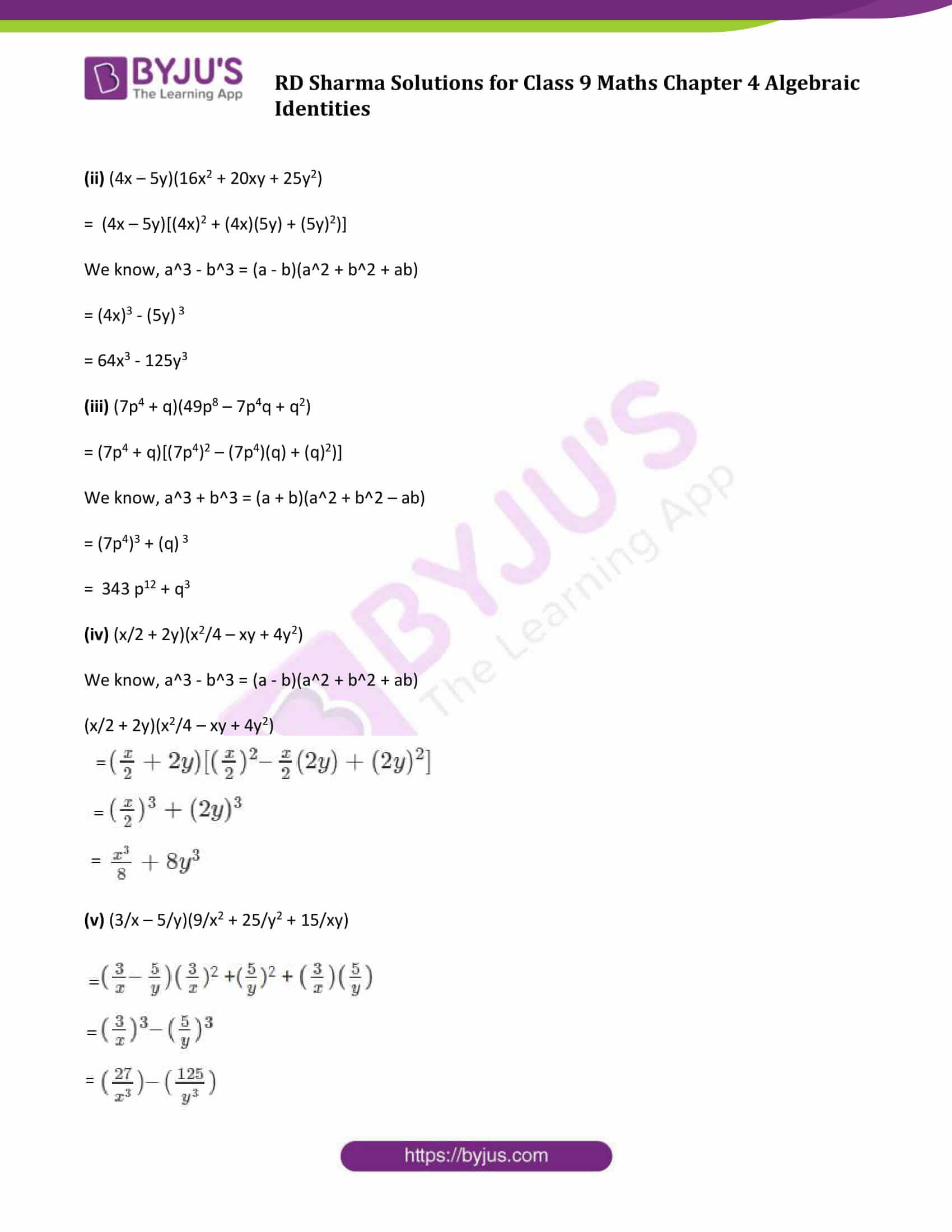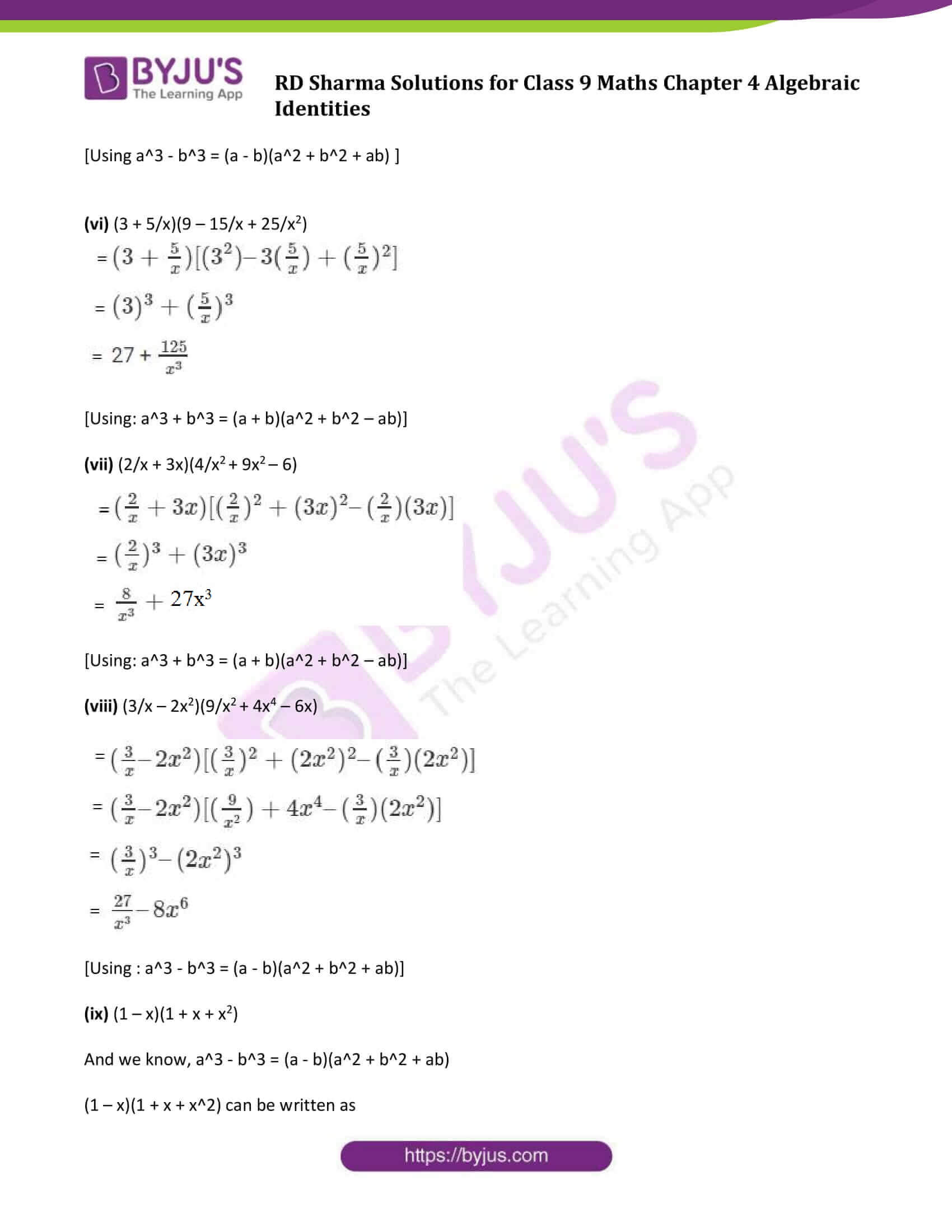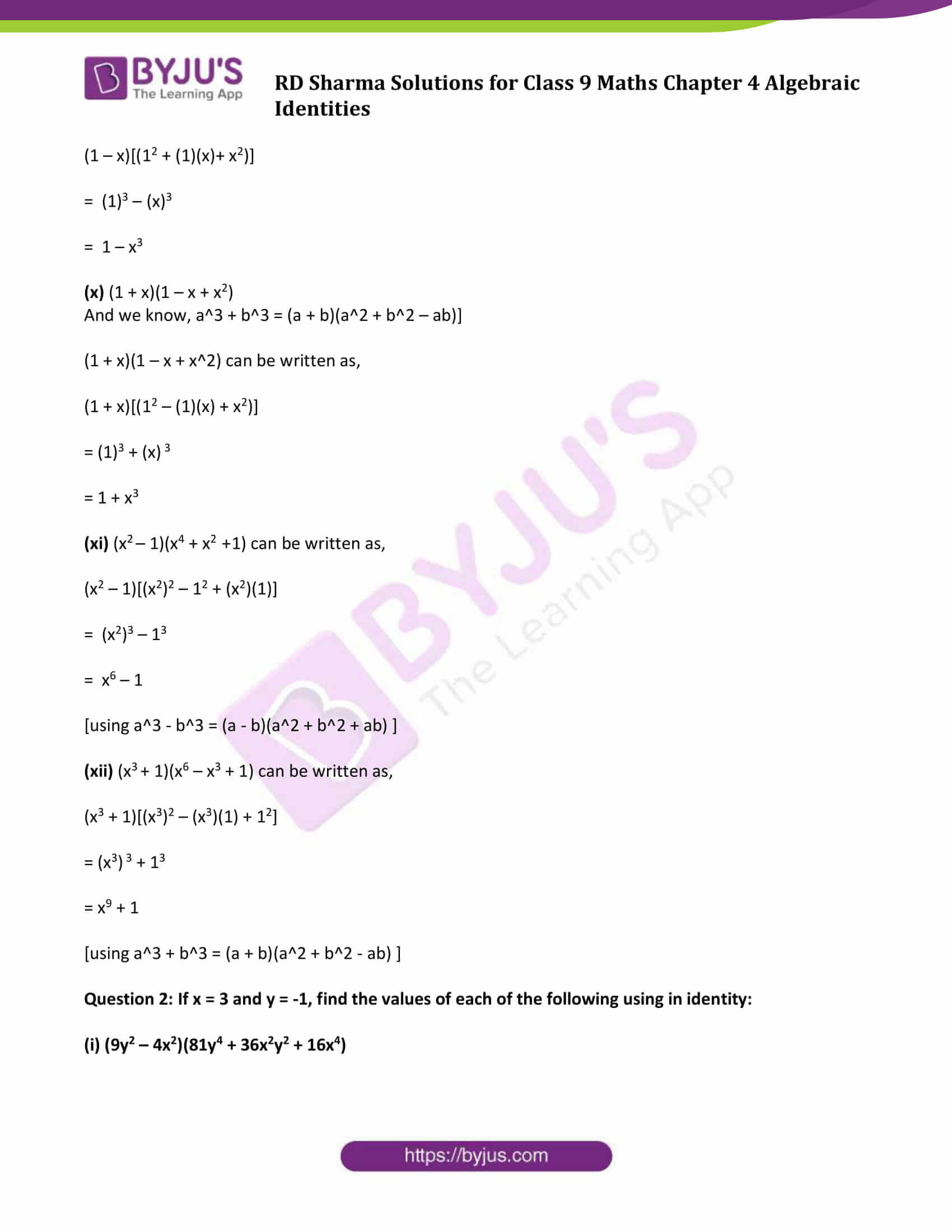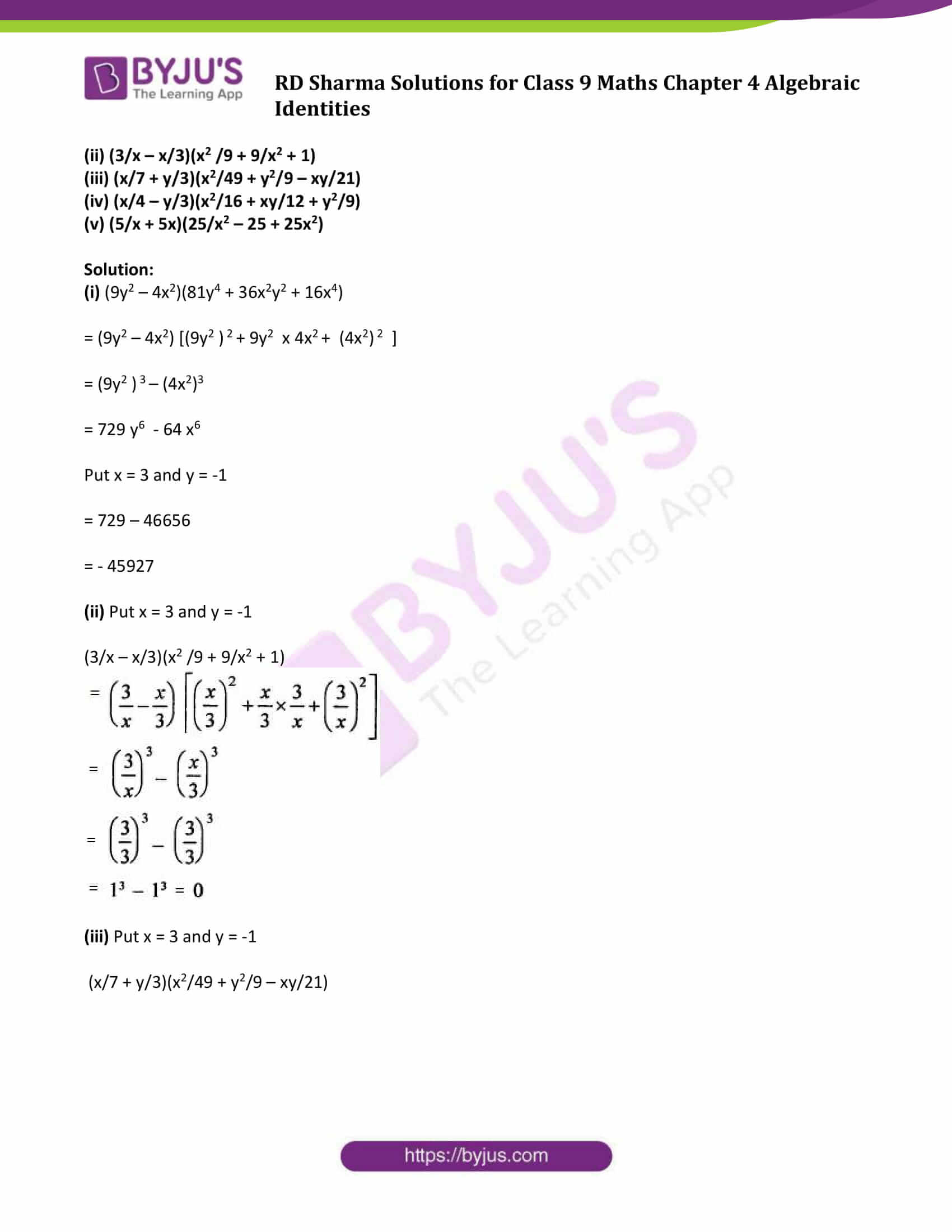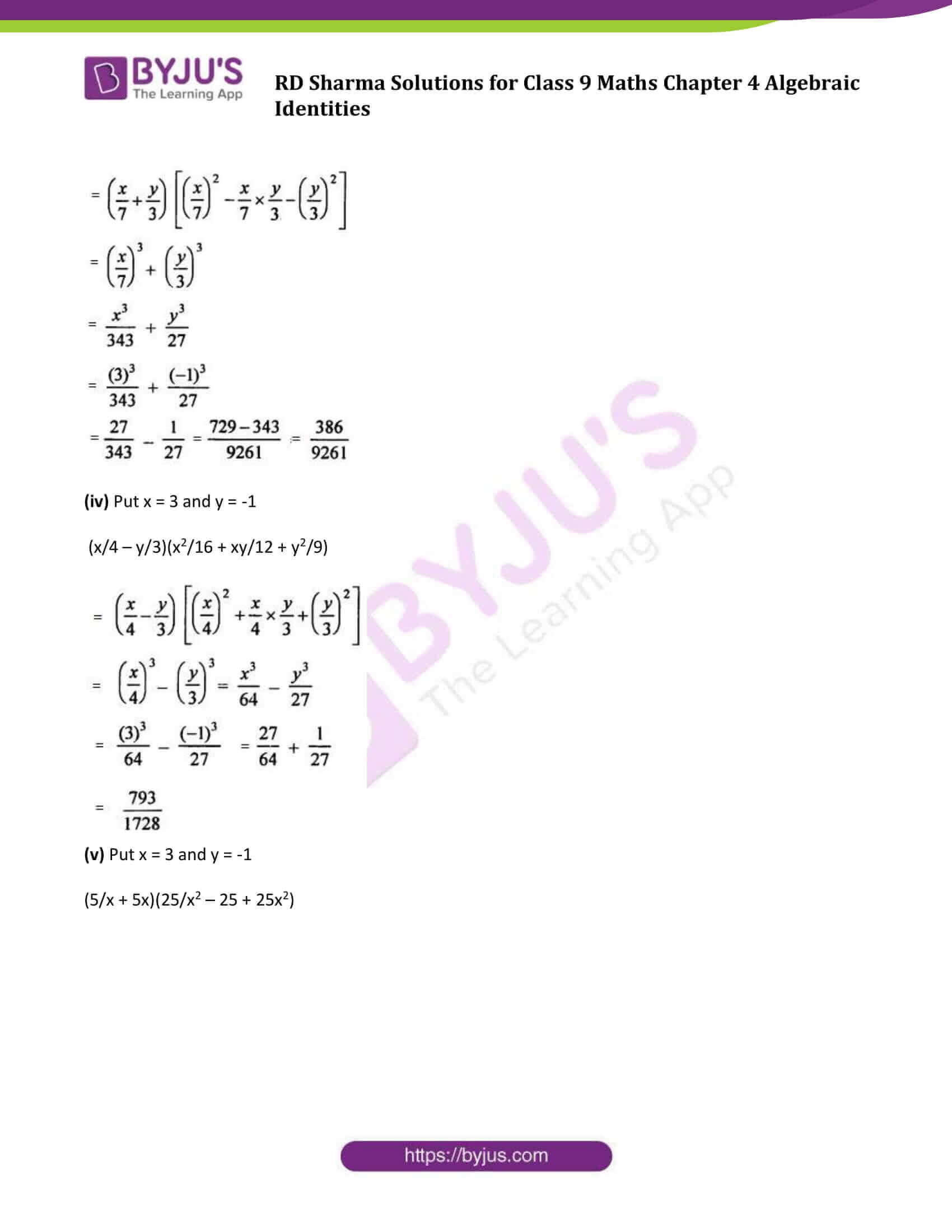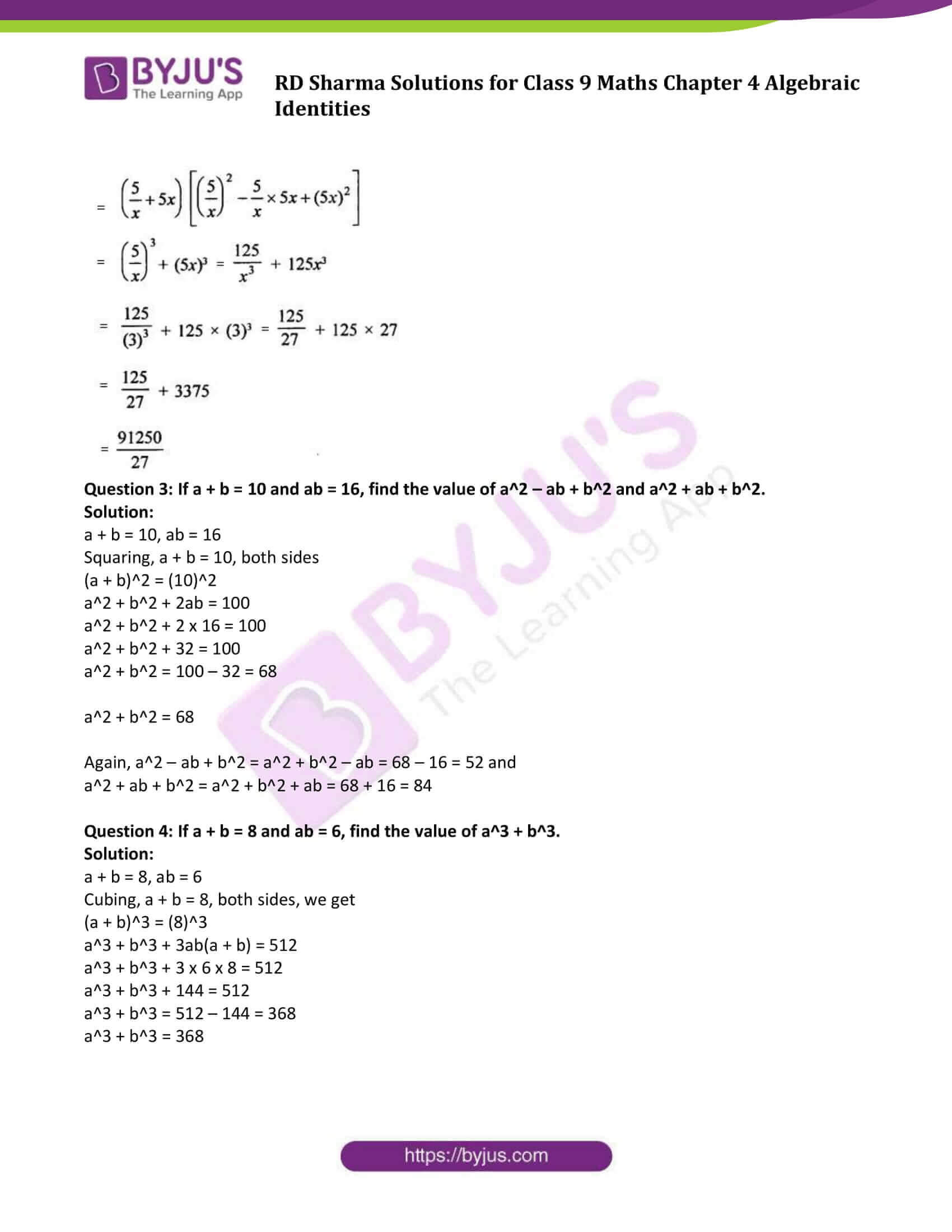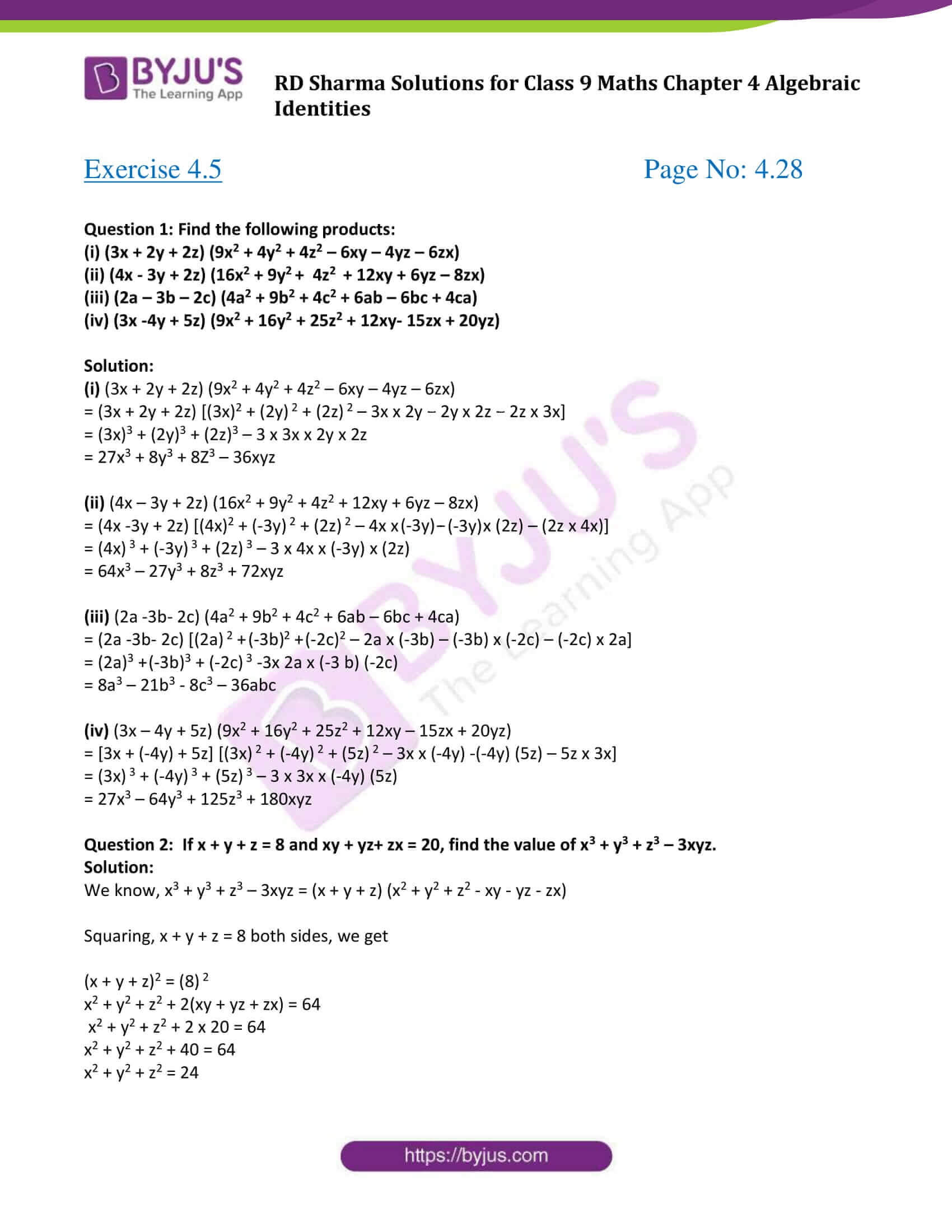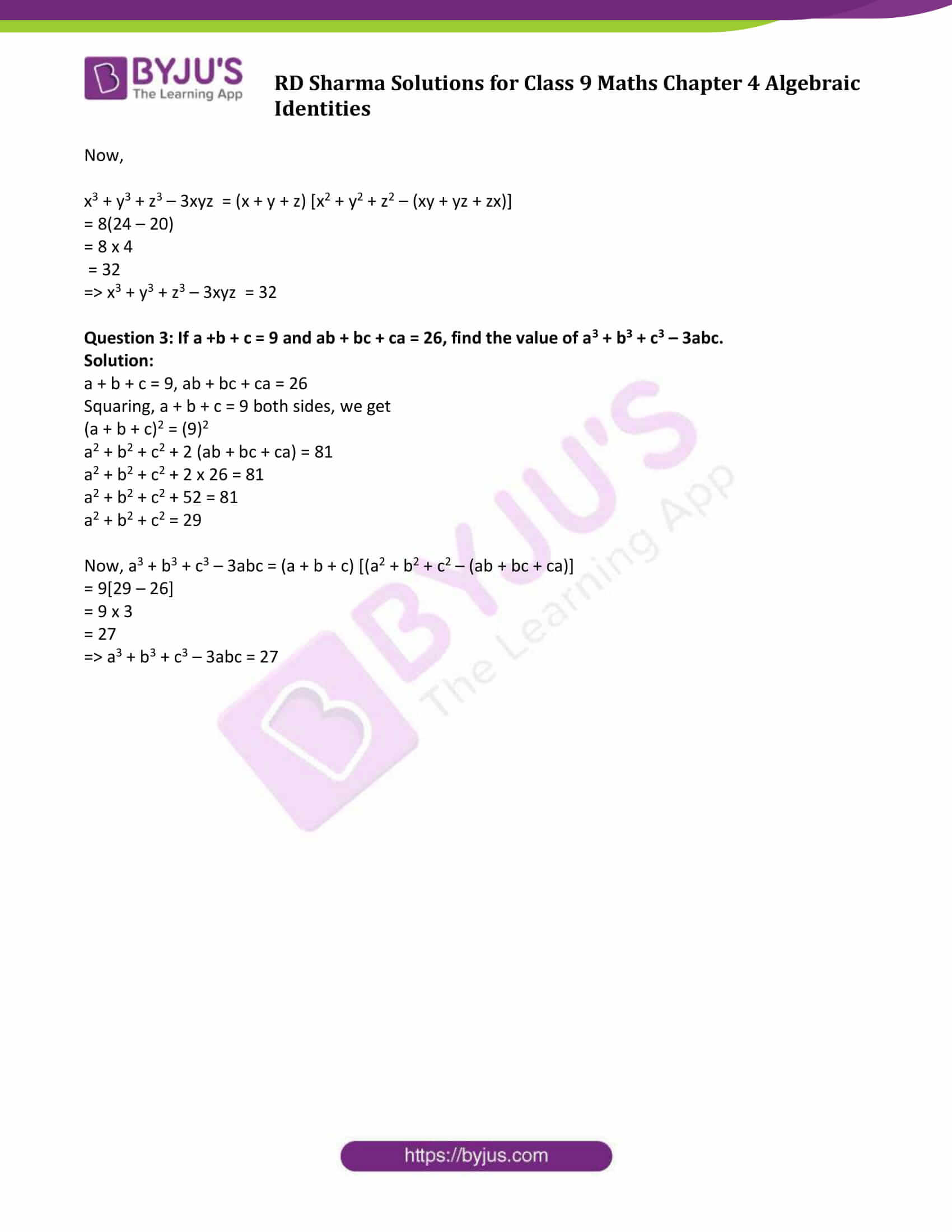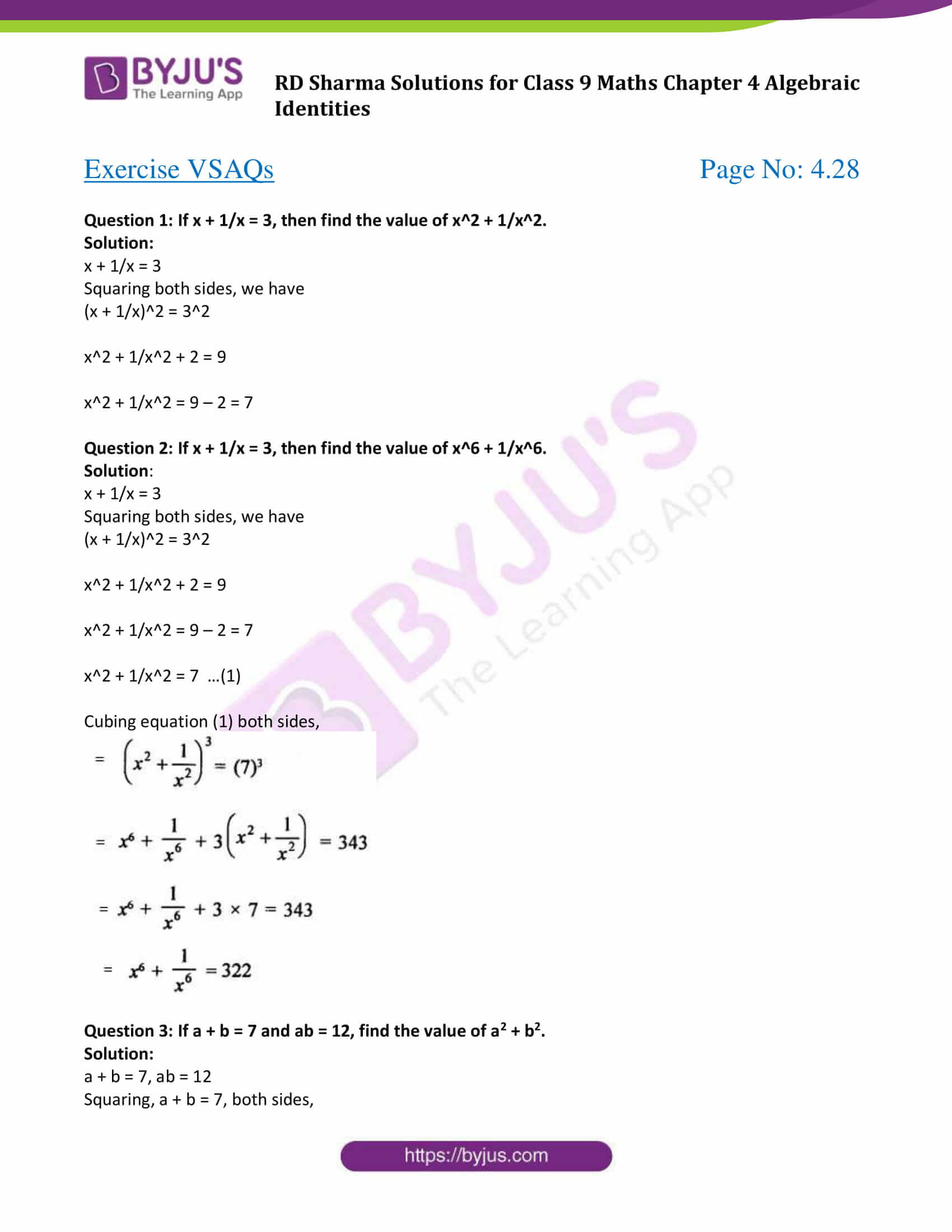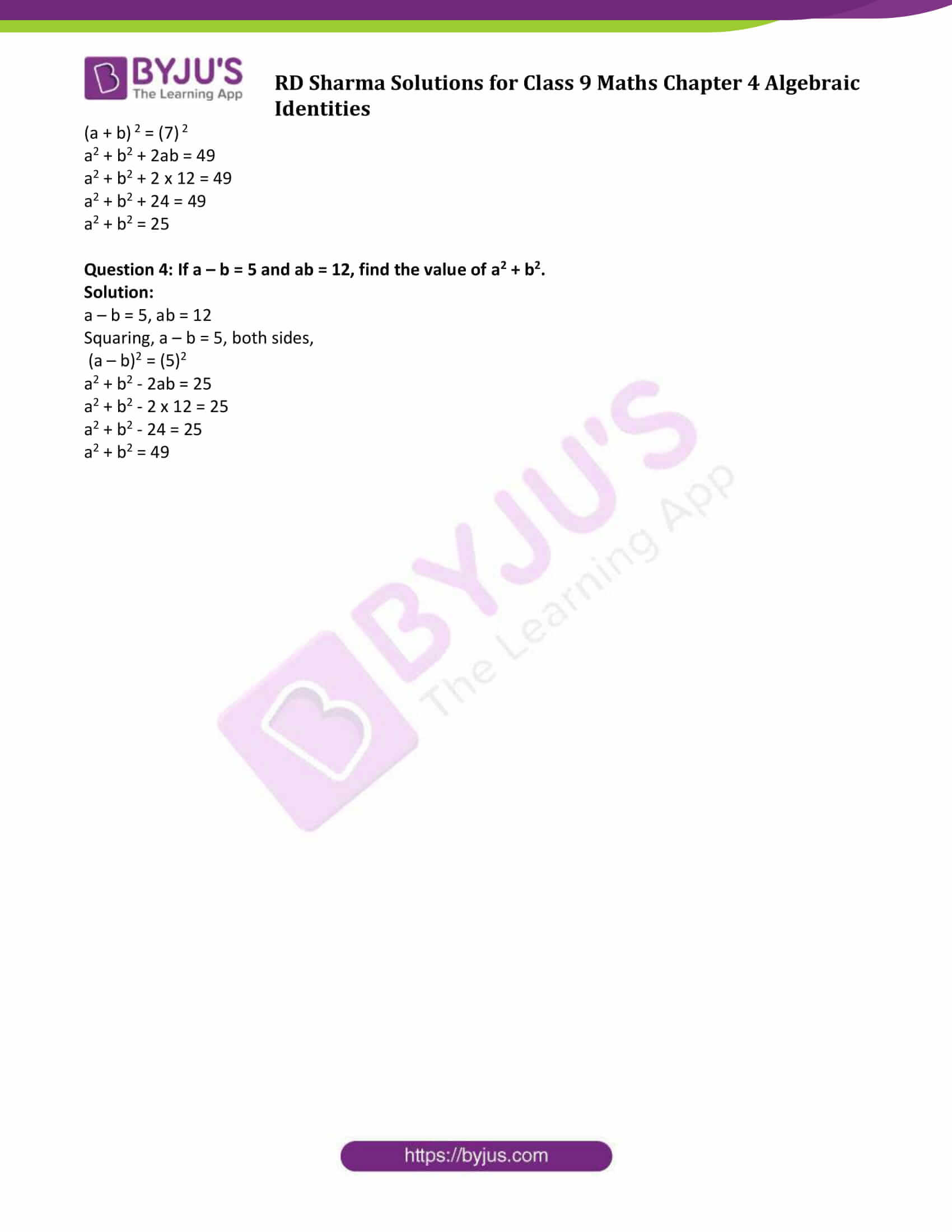### Exercise 4.1 Page No: 4.6

Question 1: Evaluate each of the following using identities:

(i) (2x – 1/x)2

(ii) (2x + y) (2x – y)

(iii) (a2b – b2a)2

(iv) (a – 0.1) (a + 0.1)

(v) (1.5.x2 – 0.3y2) (1.5x2 + 0.3y2)

Solution:

(i) (2x – 1/x)2

[Use identity: (a – b)2 = a2 + b2 – 2ab ]

(2x – 1/x)2 = (2x)2 + (1/x)2 – 2 (2x)(1/x)

= 4x2 + 1/x2 – 4

(ii) (2x + y) (2x – y)

[Use identity: (a – b)(a + b) = a2 – b2 ]

(2x + y) (2x – y) = (2x )2 – (y)2

= 4x2 – y2

(iii) (a2b – b2a)2

[Use identity: (a – b)2 = a2 + b2 – 2ab ]

(a2b – b2a)2 = (a2b) 2 + (b2a)2 – 2 (a2b)( b2a)

= a4b 2 + b4a2 – 2 a3b3

(iv) (a – 0.1) (a + 0.1)

[Use identity: (a – b)(a + b) = a2 – b2 ]

(a – 0.1) (a + 0.1) = (a)2 – (0.1)2

= (a)2 – 0.01

(v) (1.5 x2 – 0.3y2) (1.5 x2 + 0.3y2)

[Use identity: (a – b)(a + b) = a2 – b2 ]

(1.5 x2 – 0.3y2) (1.5x2 + 0.3y2) = (1.5 x2 ) 2 – (0.3y2)2

= 2.25 x4 – 0.09y4

Question 2: Evaluate each of the following using identities:

(i) (399)2

(ii) (0.98)2

(iii) 991 x 1009

(iv) 117 x 83

Solution:

(i)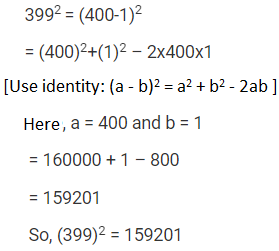(ii)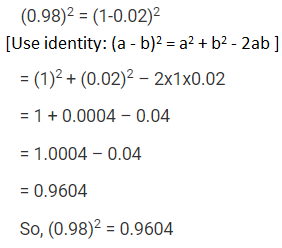(iii)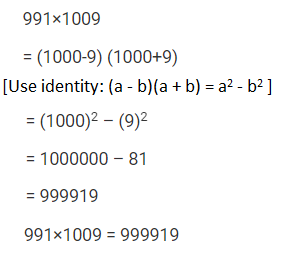(iv)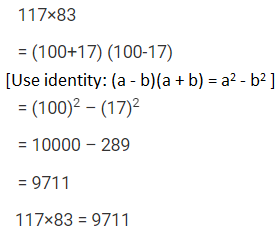Question 3: Simplify each of the following:

(i) 175 x 175 +2 x 175 x 25 + 25 x 25

(ii) 322 x 322 – 2 x 322 x 22 + 22 x 22

(iii) 0.76 x 0.76 + 2 x 0.76 x 0.24 + 0.24 x 0.24

(iv)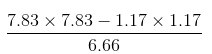Solution:

(i) 175 x 175 +2 x 175 x 25 + 25 x 25 = (175)2 + 2 (175) (25) + (25)2

= (175 + 25)2

[Because a2+ b2+2ab = (a+b)2 ]

= (200)2

= 40000

So, 175 x 175 +2 x 175 x 25 + 25 x 25 = 40000.

(ii) 322 x 322 – 2 x 322 x 22 + 22 x 22

= (322)2 – 2 x 322 x 22 + (22)2

= (322 – 22)2

[Because a2+ b2-2ab = (a-b)2]

= (300)2

= 90000

So, 322 x 322 – 2 x 322 x 22 + 22 x 22= 90000.

(iii) 0.76 x 0.76 + 2 x 0.76 x 0.24 + 0.24 x 0.24

= (0.76) 2 + 2 x 0.76 x 0.24 + (0.24) 2

= (0.76+0.24) 2

[ Because a2+ b2+2ab = (a+b)2]

= (1.00)2

= 1

So, 0.76 x 0.76 + 2 x 0.76 x 0.24 + 0.24 x 0.24 = 1.

(iv)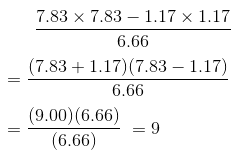Question 4: If x + 1/x = 11, find the value of x2 +1/x2.

Solution: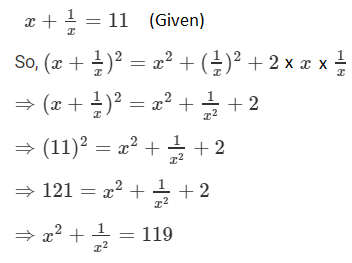Question 5: If x – 1/x = -1, find the value of x2 +1/x2.

Solution: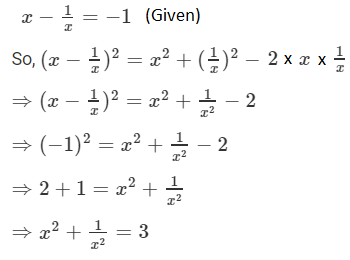### Exercise 4.2 Page No: 4.11

Question 1: Write the following in the expanded form:

(i) (a + 2b + c)2

(ii) (2a − 3b − c)2

(iii) (−3x+y+z)2

(iv) (m+2n−5p)2

(v) (2+x−2y)2

(vi) (a2 +b2 +c2) 2

(vii) (ab+bc+ca) 2

(viii) (x/y+y/z+z/x)2

(ix) (a/bc + b/ac + c/ab) 2

(x) (x+2y+4z) 2

(xi) (2x−y+z) 2

(xii) (−2x+3y+2z) 2

Solution:

Using identities:

(x + y + z)2 = x2 + y2 + z2 + 2xy + 2yz + 2xz

(i) (a + 2b + c)2

= a2 + (2b) 2 + c2 + 2a(2b) + 2ac + 2(2b)c

= a2 + 4b2 + c2 + 4ab + 2ac + 4bc

(ii) (2a − 3b − c)2

= [(2a) + (−3b) + (−c)]2

= (2a) 2 + (−3b) 2 + (−c) 2 + 2(2a)(−3b) + 2(−3b)(−c) + 2(2a)(−c)

= 4a2 + 9b2 + c2 − 12ab + 6bc − 4ca

(iii) (−3x+y+z)2

= [(−3x) 2 + y2 + z2 + 2(−3x)y + 2yz + 2(−3x)z

= 9x2 + y2 + z2 − 6xy + 2yz − 6xz

(iv) (m+2n−5p)2

= m2 + (2n) 2 + (−5p) 2 + 2m × 2n + (2×2n×−5p) + 2m × −5p

= m2 + 4n2 + 25p2 + 4mn − 20np − 10pm

(v) (2+x−2y)2

= 22 + x2 + (−2y) 2 + 2(2)(x) + 2(x)(−2y) + 2(2)(−2y)

= 4 + x2 + 4y2 + 4 x − 4xy − 8y

(vi) (a2 +b2 +c2) 2

= (a2) 2 + (b2) 2 + (c2 ) 2 + 2a2 b2 + 2b2c2 + 2a2c2

= a4 + b4 + c4 + 2a2 b2 + 2b2 c2 + 2c2 a2

(vii) (ab+bc+ca) 2

= (ab)2 + (bc) 2 + (ca) 2 + 2(ab)(bc) + 2(bc)(ca) + 2(ab)(ca)

= a2 b2 + b2c2 + c2 a2 + 2(ac)b2 + 2(ab)(c) 2 + 2(bc)(a) 2

(viii) (x/y+y/z+z/x)2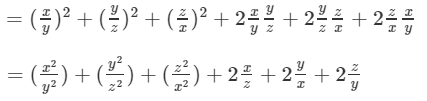(ix) (a/bc + b/ac + c/ab) 2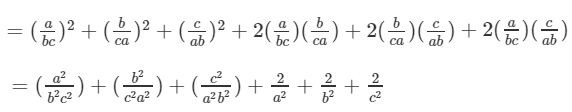(x) (x+2y+4z) 2

= x2 + (2y) 2 + (4z) 2 + (2x)(2y) + 2(2y)(4z) + 2x(4z)

= x2 + 4y2 + 16z2 + 4xy + 16yz + 8xz

(xi) (2x−y+z) 2

= (2x) 2 + (−y) 2 + (z) 2 + 2(2x)(−y) + 2(−y)(z) + 2(2x)(z)

= 4x2 + y2 + z2 − 4xy−2yz+4xz

(xii) (−2x+3y+2z) 2

= (−2x) 2 + (3y) 2 + ( 2z) 2 + 2(−2x)(3y)+2(3y)(2z)+2(−2x)(2z)

= 4x2 + 9y2 + 4z2 −12xy+12yz−8xz

Question 2: Simplify

(i) (a + b + c)2 + (a − b + c) 2

(ii) (a + b + c)2 − (a − b + c) 2

(iii) (a + b + c)2 + (a – b + c) 2 + (a + b − c) 2

(iv) (2x + p − c)2 − (2x − p + c) 2

(v) (x2 + y2 − z2) 2 − (x2 − y2 + z2) 2

Solution:

(i) (a + b + c)2 + (a − b + c) 2

= (a2 + b2 + c2 + 2ab+2bc+2ca) + (a2 + (−b) 2 + c2 −2ab−2bc+2ca)

= 2a2 + 2 b2 + 2c2 + 4ca

(ii) (a + b + c)2 − (a − b + c) 2

= (a2 + b2 + c2 + 2ab+2bc+2ca) − (a2 + (−b) 2 + c2 −2ab−2bc+2ca)

= a2 + b2 + c2 + 2ab + 2bc + 2ca − a2 − b2 − c2 + 2ab + 2bc − 2ca

= 4ab + 4bc

(iii) (a + b + c)2 + (a – b + c) 2 + (a + b − c) 2

= a2 + b2 + c2 + 2ab + 2bc + 2ca + (a2 + b2 + (c) 2 − 2ab − 2cb + 2ca) + (a2 + b2 + c2 + 2ab − 2bc – 2ca)

= 3 a2 + 3b2 + 3c2 + 2ab − 2bc + 2ca

(iv) (2x + p − c)2 − (2x − p + c) 2

= [4x2 + p2 + c2 + 4xp − 2pc − 4xc] − [4x2 + p2 + c2 − 4xp− 2pc + 4xc]

= 4x2 + p2 + c2 + 4xp − 2pc − 4cx − 4x2 − p2 − c2 + 4xp + 2pc− 4cx

= 8xp − 8xc

= 8(xp − xc)

(v) (x2 + y2 − z2) 2 − (x2 − y2 + z2) 2

= (x2 + y2 + (−z) 2) 2 − (x2 − y2 + z2) 2

= [x4 + y4 + z4 + 2x2y2 – 2y2z 2 – 2x2z2 − [x4 + y4 + z4 − 2x2y2 − 2y2z2 + 2x2z2]

= 4x2y2 – 4z2x2

Question 3: If a + b + c = 0 and a2 + b2 + c2 = 16, find the value of ab + bc + ca.

Solution:

a + b + c = 0 and a2 + b2 + c2 = 16 (given)

Choose a + b + c = 0

Squaring both sides,

(a + b + c)2 = 0

a2 + b2 + c2 + 2(ab + bc + ca) = 0

16 + 2(ab + bc + c) = 0

2(ab + bc + ca) = -16

ab + bc + ca = -16/2 = -8

or ab + bc + ca = -8

### Exercise 4.3 Page No: 4.19

Question 1: Find the cube of each of the following binomial expressions:

(i) (1/x + y/3)

(ii) (3/x – 2/x2)

(iii) (2x + 3/x)

(iv) (4 – 1/3x)

Solution:

[Using identities: (a + b)3 = a3 + b3 + 3ab(a + b) and (a – b)3 = a3 – b3 – 3ab(a – b) ]

(i)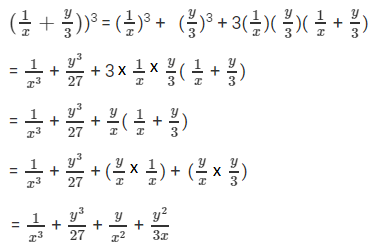(ii)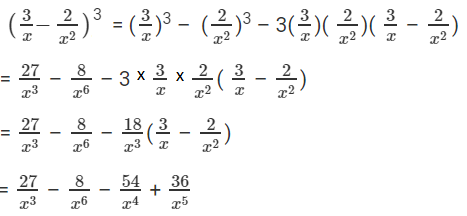(iii)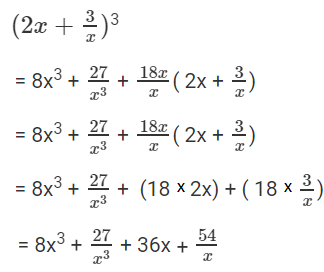(iv)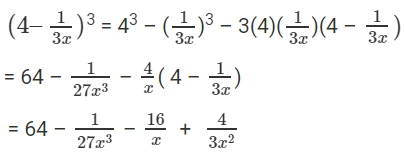Question 2: Simplify each of the following:

(i) (x + 3)3 + (x – 3) 3

(ii) (x/2 + y/3) 3 – (x/2 – y/3) 3

(iii) (x + 2/x) 3 + (x – 2/x) 3

(iv) (2x – 5y) 3 – (2x + 5y) 3

Solution:

[Using identities:

a3 + b3 = (a + b)(a2 + b2 – ab)

a3 – b3 = (a – b)(a2 + b2 + ab)

(a + b)(a-b) = a2 – b2

(a + b)2 = a2 + b2 + 2ab and

(a – b)2 = a2 + b2 – 2ab]

(i) (x + 3)3 + (x – 3) 3

Here a = (x + 3), b = (x – 3)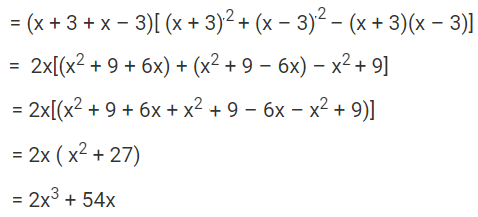(ii) (x/2 + y/3) 3 – (x/2 – y/3) 3

Here a = (x/2 + y/3) and b = (x/2 – y/3)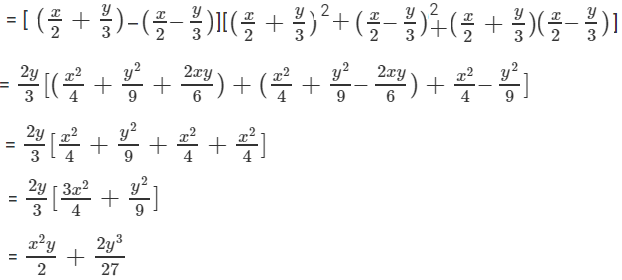(iii) (x + 2/x) 3 + (x – 2/x) 3

Here a = (x + 2/x) and b = (x – 2/x)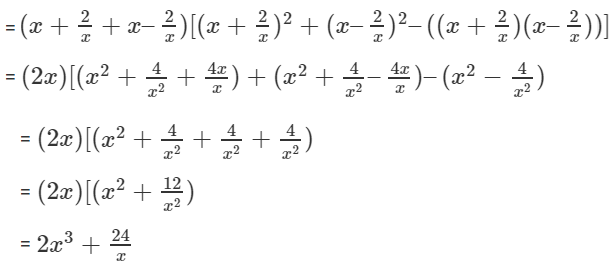(iv) (2x – 5y) 3 – (2x + 5y) 3

Here a = (2x – 5y) and b = 2x + 5y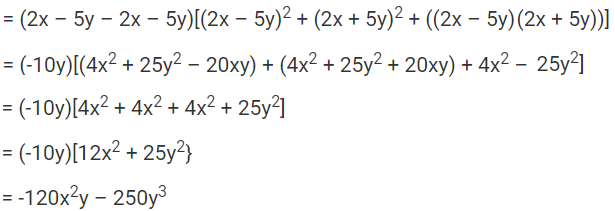Question 3: If a + b = 10 and ab = 21, find the value of a3 + b3.

Solution:

a + b = 10, ab = 21 (given)

Choose a + b = 10

Cubing both sides,

(a + b)3 = (10)3

a3 + b3 + 3ab(a + b) = 1000

a3 + b3 + 3 x 21 x 10 = 1000 (using given values)

a3 + b3 + 630 = 1000

a3 + b3 = 1000 – 630 = 370

or a3 + b3 = 370

Question 4: If a – b = 4 and ab = 21, find the value of a3 – b3.

Solution:

a – b = 4, ab= 21 (given)

Choose a – b = 4

Cubing both sides,

(a – b)3 = (4)3

a3 – b3 – 3ab (a – b) = 64

a3 – b3 – 3 × 21 x 4 = 64 (using given values)

a3 – b3 – 252 = 64

a3 – b3 = 64 + 252

= 316

Or a3 – b3 = 316

Question 5: If x + 1/x = 5, find the value of x3 + 1/x3 .

Solution:

Given: x + 1/x = 5

Apply Cube on x + 1/x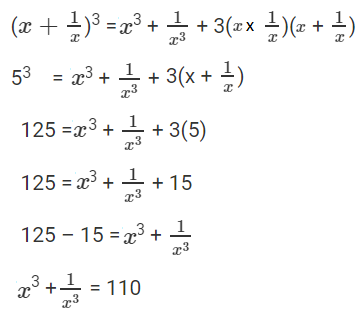Question 6: If x – 1/x = 7, find the value of x3 – 1/x3 .

Solution:

Given: x – 1/x = 7

Apply Cube on x – 1/x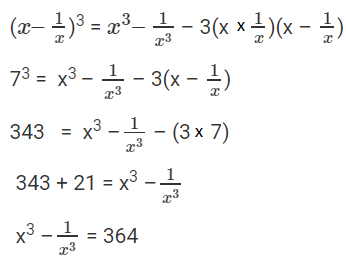Question 7: If x – 1/x = 5, find the value of x3 – 1/x3 .

Solution:

Given: x – 1/x = 5

Apply Cube on x – 1/x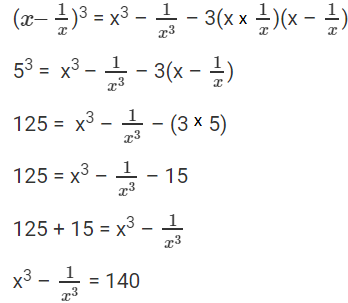Question 8: If (x2 + 1/x2) = 51, find the value of x3 – 1/x3.

Solution:

We know that: (x – y)2 = x2 + y2 – 2xy

Replace y with 1/x, we get

(x – 1/x)2 = x2 + 1/x2 – 2

Since (x2 + 1/x2) = 51 (given)

(x – 1/x)2 = 51 – 2 = 49

or (x – 1/x) = ±7

Now, Find x3 – 1/x3

We know that, x3 – y3 = (x – y)(x2 + y2 + xy)

Replace y with 1/x, we get

x3 – 1/x3 = (x – 1/x)(x2 + 1/x2 + 1)

Use (x – 1/x) = 7 and (x2 + 1/x2) = 51

x3 – 1/x3 = 7 x 52 = 364

x3 – 1/x3 = 364

Question 9: If (x2 + 1/x2) = 98, find the value of x3 + 1/x3.

Solution:

We know that: (x + y)2 = x2 + y2 + 2xy

Replace y with 1/x, we get

(x + 1/x)2 = x2 + 1/x2 + 2

Since (x2 + 1/x2) = 98 (given)

(x + 1/x)2 = 98 + 2 = 100

or (x + 1/x) = ±10

Now, Find x3 + 1/x3

We know that, x3 + y3 = (x + y)(x2 + y2 – xy)

Replace y with 1/x, we get

x3 + 1/x3 = (x + 1/x)(x2 + 1/x2 – 1)

Use (x + 1/x) = 10 and (x2 + 1/x2) = 98

x3 + 1/x3 = 10 x 97 = 970

x3 + 1/x3 = 970

Question 10: If 2x + 3y = 13 and xy = 6, find the value of 8x3 + 27y3.

Solution:

Given: 2x + 3y = 13, xy = 6

Cubing 2x + 3y = 13 both sides, we get

(2x + 3y)3 = (13)3

(2x)3 + (3y) 3 + 3( 2x )(3y) (2x + 3y) = 2197

8x3 + 27y3 + 18xy(2x + 3y) = 2197

8x3 + 27y3 + 18 x 6 x 13 = 2197

8x3 + 27y3 + 1404 = 2197

8x3 + 27y3 = 2197 – 1404 = 793

8x3 + 27y3 = 793

Question 11: If 3x – 2y= 11 and xy = 12, find the value of 27x3 – 8y3.

Solution:

Given: 3x – 2y = 11 and xy = 12

Cubing 3x – 2y = 11 both sides, we get

(3x – 2y)3 = (11)3

(3x)3 – (2y)3 – 3 ( 3x)( 2y) (3x – 2y) =1331

27x3 – 8y3 – 18xy(3x -2y) =1331

27x3 – 8y3 – 18 x 12 x 11 = 1331

27x3 – 8y3 – 2376 = 1331

27x3 – 8y3 = 1331 + 2376 = 3707

27x3 – 8y3 = 3707

### Exercise 4.4 Page No: 4.23

Question 1: Find the following products:

(i) (3x + 2y)(9x2 – 6xy + 4y2)

(ii) (4x – 5y)(16x2 + 20xy + 25y2)

(iii) (7p4 + q)(49p8 – 7p4q + q2)

(iv) (x/2 + 2y)(x2/4 – xy + 4y2)

(v) (3/x – 5/y)(9/x2 + 25/y2 + 15/xy)

(vi) (3 + 5/x)(9 – 15/x + 25/x2)

(vii) (2/x + 3x)(4/x2 + 9x2 – 6)

(viii) (3/x – 2x2)(9/x2 + 4x4 – 6x)

(ix) (1 – x)(1 + x + x2)

(x) (1 + x)(1 – x + x2)

(xi) (x2 – 1)(x4 + x2 +1)

(xii) (x3 + 1)(x6 – x3 + 1)

Solution:

(i) (3x + 2y)(9x2 – 6xy + 4y2)

= (3x + 2y)[(3x)2 – (3x)(2y) + (2y)2)]

We know, a3 + b3 = (a + b)(a2 + b2 – ab)

= (3x)3 + (2y) 3

= 27x3 + 8y3

(ii) (4x – 5y)(16x2 + 20xy + 25y2)

= (4x – 5y)[(4x)2 + (4x)(5y) + (5y)2)]

We know, a3 – b3 = (a – b)(a2 + b2 + ab)

= (4x)3 – (5y) 3

= 64x3 – 125y3

(iii) (7p4 + q)(49p8 – 7p4q + q2)

= (7p4 + q)[(7p4)2 – (7p4)(q) + (q)2)]

We know, a3 + b3 = (a + b)(a2 + b2 – ab)

= (7p4)3 + (q) 3

= 343 p12 + q3

(iv) (x/2 + 2y)(x2/4 – xy + 4y2)

We know, a3 – b3 = (a – b)(a2 + b2 + ab)

(x/2 + 2y)(x2/4 – xy + 4y2)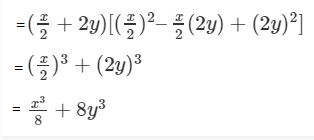(v) (3/x – 5/y)(9/x2 + 25/y2 + 15/xy)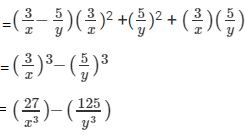[Using a3 – b3 = (a – b)(a2 + b2 + ab) ]

(vi) (3 + 5/x)(9 – 15/x + 25/x2)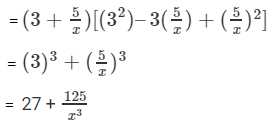[Using: a3 + b3 = (a + b)(a2 + b2 – ab)]

(vii) (2/x + 3x)(4/x2 + 9x2 – 6)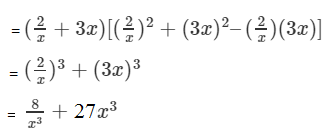[Using: a3 + b3 = (a + b)(a2 + b2 – ab)]

(viii) (3/x – 2x2)(9/x2 + 4x4 – 6x)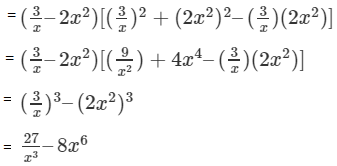[Using : a3 – b3 = (a – b)(a2 + b2 + ab)]

(ix) (1 – x)(1 + x + x2)

And we know, a3 – b3 = (a – b)(a2 + b2 + ab)

(1 – x)(1 + x + x2) can be written as

(1 – x)[(12 + (1)(x)+ x2)]

= (1)3 – (x)3

= 1 – x3

(x) (1 + x)(1 – x + x2)

And we know, a3 + b3 = (a + b)(a2 + b2 – ab)]

(1 + x)(1 – x + x2) can be written as,

(1 + x)[(12 – (1)(x) + x2)]

= (1)3 + (x) 3

= 1 + x3

(xi) (x2 – 1)(x4 + x2 +1) can be written as,

(x2 – 1)[(x2)2 – 12 + (x2)(1)]

= (x2)3 – 13

= x6 – 1

[using a3 – b3 = (a – b)(a2 + b2 + ab) ]

(xii) (x3 + 1)(x6 – x3 + 1) can be written as,

(x3 + 1)[(x3)2 – (x3)(1) + 12]

= (x3) 3 + 13

= x9 + 1

[using a3 + b3 = (a + b)(a2 + b2 – ab) ]

Question 2: If x = 3 and y = -1, find the values of each of the following using in identity:

(i) (9y2 – 4x2)(81y4 + 36x2y2 + 16x4)

(ii) (3/x – x/3)(x2 /9 + 9/x2 + 1)

(iii) (x/7 + y/3)(x2/49 + y2/9 – xy/21)

(iv) (x/4 – y/3)(x2/16 + xy/12 + y2/9)
(v) (5/x + 5x)(25/x2 – 25 + 25x2)

Solution:

(i) (9y2 – 4x2)(81y4 + 36x2y2 + 16x4)

= (9y2 – 4x2) [(9y2 ) 2 + 9y2 x 4x2 + (4x2) 2 ]

= (9y2 ) 3 – (4x2)3

= 729 y6 – 64 x6

Put x = 3 and y = -1

= 729 – 46656

= – 45927

(ii) Put x = 3 and y = -1

(3/x – x/3)(x2 /9 + 9/x2 + 1)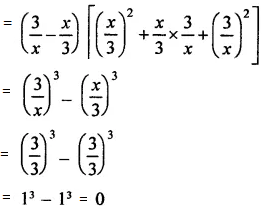(iii) Put x = 3 and y = -1

(x/7 + y/3)(x2/49 + y2/9 – xy/21)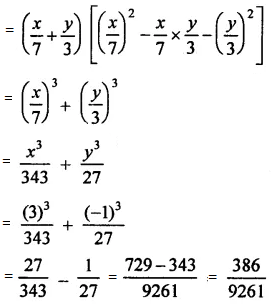(iv) Put x = 3 and y = -1

(x/4 – y/3)(x2/16 + xy/12 + y2/9)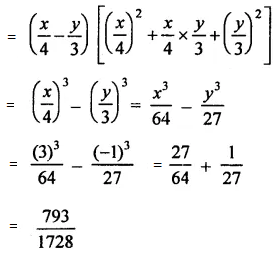(v) Put x = 3 and y = -1

(5/x + 5x)(25/x2 – 25 + 25x2)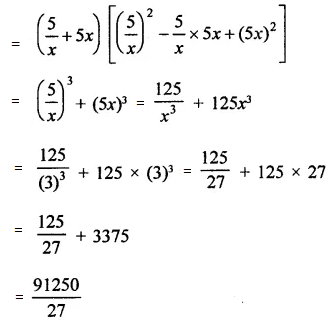Question 3: If a + b = 10 and ab = 16, find the value of a2 – ab + b2 and a2 + ab + b2.

Solution:

a + b = 10, ab = 16

Squaring, a + b = 10, both sides

(a + b)2 = (10)2

a2 + b2 + 2ab = 100

a2 + b2 + 2 x 16 = 100

a2 + b2 + 32 = 100

a2 + b2 = 100 – 32 = 68

a2 + b2 = 68

Again, a2 – ab + b2 = a2 + b2 – ab = 68 – 16 = 52 and

a2 + ab + b2 = a2 + b2 + ab = 68 + 16 = 84

Question 4: If a + b = 8 and ab = 6, find the value of a3 + b3.

Solution:

a + b = 8, ab = 6

Cubing, a + b = 8, both sides, we get

(a + b)3 = (8)3

a3 + b3 + 3ab(a + b) = 512

a3 + b3 + 3 x 6 x 8 = 512

a3 + b3 + 144 = 512

a3 + b3 = 512 – 144 = 368

a3 + b3 = 368

### Exercise 4.5 Page No: 4.28

Question 1: Find the following products:

(i) (3x + 2y + 2z) (9x2 + 4y2 + 4z2 – 6xy – 4yz – 6zx)

(ii) (4x – 3y + 2z) (16x2 + 9y2 + 4z2 + 12xy + 6yz – 8zx)

(iii) (2a – 3b – 2c) (4a2 + 9b2 + 4c2 + 6ab – 6bc + 4ca)

(iv) (3x -4y + 5z) (9x2 + 16y2 + 25z2 + 12xy- 15zx + 20yz)

Solution:

(i) (3x + 2y + 2z) (9x2 + 4y2 + 4z2 – 6xy – 4yz – 6zx)

= (3x + 2y + 2z) [(3x)2 + (2y) 2 + (2z) 2 – 3x x 2y – 2y x 2z – 2z x 3x]

= (3x)3 + (2y)3 + (2z)3 – 3 x 3x x 2y x 2z

= 27x3 + 8y3 + 8Z3 – 36xyz

(ii) (4x – 3y + 2z) (16x2 + 9y2 + 4z2 + 12xy + 6yz – 8zx)

= (4x -3y + 2z) [(4x)2 + (-3y) 2 + (2z) 2 – 4x x (-3y) – (-3y) x (2z) – (2z x 4x)]

= (4x) 3 + (-3y) 3 + (2z) 3 – 3 x 4x x (-3y) x (2z)

= 64x3 – 27y3 + 8z3 + 72xyz

(iii) (2a -3b- 2c) (4a2 + 9b2 + 4c2 + 6ab – 6bc + 4ca)

= (2a -3b- 2c) [(2a) 2 + (-3b) 2 + (-2c) 2 – 2a x (-3b) – (-3b) x (-2c) – (-2c) x 2a]

= (2a)3 + (-3b) 3 + (-2c) 3 -3x 2a x (-3 b) (-2c)

= 8a3 – 21b3 – 8c3 – 36abc

(iv) (3x – 4y + 5z) (9x2 + 16y2 + 25z2 + 12xy – 15zx + 20yz)

= [3x + (-4y) + 5z] [(3x) 2 + (-4y) 2 + (5z) 2 – 3x x (-4y) -(-4y) (5z) – 5z x 3x]

= (3x) 3 + (-4y) 3 + (5z) 3 – 3 x 3x x (-4y) (5z)

= 27x3 – 64y3 + 125z3 + 180xyz

Question 2: If x + y + z = 8 and xy + yz+ zx = 20, find the value of x3 + y3 + z3 – 3xyz.

Solution:

We know, x3 + y3 + z3 – 3xyz = (x + y + z) (x2 + y2 + z2 – xy – yz – zx)

Squaring, x + y + z = 8 both sides, we get

(x + y + z)2 = (8) 2

x2 + y2 + z2 + 2(xy + yz + zx) = 64

x2 + y2 + z2 + 2 x 20 = 64

x2 + y2 + z2 + 40 = 64

x2 + y2 + z2 = 24

Now,

x3 + y3 + z3 – 3xyz = (x + y + z) [x2 + y2 + z2 – (xy + yz + zx)]

= 8(24 – 20)

= 8 x 4

= 32

⇒ x3 + y3 + z3 – 3xyz = 32

Question 3: If a +b + c = 9 and ab + bc + ca = 26, find the value of a3 + b3 + c3 – 3abc.

Solution:

a + b + c = 9, ab + bc + ca = 26

Squaring, a + b + c = 9 both sides, we get

(a + b + c)2 = (9)2

a2 + b2 + c2 + 2 (ab + bc + ca) = 81

a2 + b2 + c2 + 2 x 26 = 81

a2 + b2 + c2 + 52 = 81

a2 + b2 + c2 = 29

Now, a3 + b3 + c3 – 3abc = (a + b + c) [(a2 + b2 + c2 – (ab + bc + ca)]

= 9[29 – 26]

= 9 x 3

= 27

⇒ a3 + b3 + c3 – 3abc = 27

### Exercise VSAQs Page No: 4.28

Question 1: If x + 1/x = 3, then find the value of x2 + 1/x2.

Solution:

x + 1/x = 3

Squaring both sides, we have

(x + 1/x)2 = 32

x2 + 1/x2 + 2 = 9

x2 + 1/x2 = 9 – 2 = 7

Question 2: If x + 1/x = 3, then find the value of x^6 + 1/x^6.

Solution:

x + 1/x = 3

Squaring both sides, we have

(x + 1/x)2 = 32

x2 + 1/x2 + 2 = 9

x2 + 1/x2 = 9 – 2 = 7

x2 + 1/x2 = 7 …(1)

Cubing equation (1) both sides,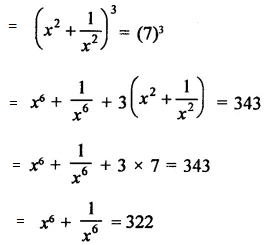Question 3: If a + b = 7 and ab = 12, find the value of a2 + b2.

Solution:

a + b = 7, ab = 12

Squaring, a + b = 7, both sides,

(a + b) 2 = (7) 2

a2 + b2 + 2ab = 49

a2 + b2 + 2 x 12 = 49

a2 + b2 + 24 = 49

a2 + b2 = 25

Question 4: If a – b = 5 and ab = 12, find the value of a2 + b2.

Solution:

a – b = 5, ab = 12

Squaring, a – b = 5, both sides,

(a – b)2 = (5)2

a2 + b2 – 2ab = 25

a2 + b2 – 2 x 12 = 25

a2 + b2 – 24 = 25

a2 + b2 = 49

### RD Sharma Solutions for Class 9 Maths Chapter 4 Algebraic Identities

In the 4th Chapter of Class 9 RD Sharma Solutions students will study important identities as listed below:

• Algebraic Identities Introduction
• Identity for the square of a trinomial
• Sum and difference of cubes Identity

These books are widely used by the students who wish to score high in board exams. For RD Sharma Class 9 Maths Solutions, you can visit the BYJU’S website, there you can get step by step answers to all the questions provided in RD Sharma textbook.

## Frequently Asked Questions on RD Sharma Solutions for Class 9 Maths Chapter 4

### Write the key benefits of RD Sharma Solutions for Class 9 Maths Chapter 4.

The key benefits of RD Sharma Solutions are as follows:
1. The solutions provided in RD Sharma Solutions for Class 9 Maths Chapter 4 are offered in a step by step approach for a comfortable and better understanding of concepts.
2. It also provides explanatory diagrams and tables for effective learning, which boost skills among students which are crucial for exams.
3. These solutions facilitate the students to build a good knowledge of basics as well as advanced mathematical concepts.
4. It also helps students to understand the concepts in an effective manner.

### How is RD Sharma Solutions for Class 9 Maths Chapter 4 helpful for Class 9 students?

The RD Sharma Solutions for Class 9 Maths Chapter 4 help students to clear their doubts and prepare themselves for the exam in a better way. Students who aspire to gain proficiency in solving the problems are suggested to practice RD Sharma Solutions on a regular basis. Experts at BYJU’S have designed the solutions to help students to excel not only in board exams but also for other competitive exams.

### How to score high marks using RD Sharma Solutions for Class 9 Maths Chapter 4 in board exams?

Students are advised to follow the RD Sharma Solutions for Class 9 Chapter 4 on a regular basis to get in-depth knowledge about all the concepts covered in the syllabus. These RD Sharma Solutions for Class 9 are prepared by our BYJU’S expert team, focusing completely on accuracy. Using these solutions while practising textbook questions helps students to clear their confusion and score high marks in board exams.